# 1 Applying a VAR model to macroeconomic data

The first exercise makes use of two well known time series variables for output and unemployment in the United States. This example is contained in the file `T7-varBQus.R`. To start off we can clear all the variables from the current environment and close all the plots.

``````rm(list = ls())
graphics.off()``````

Thereafter, we will need to make use of the `vars` and `tsm` packages that were previosuly installed. To acccess these routines we make use of the `library` command.

``````library(tsm)
library(vars)
library(mFilter)``````

If you need install reinstall these packages run the following routines: `library(devtools)`, `devtools::install_github("KevinKotze/tsm")`, `install.packages("vars")`.

As this data is contained in a `.csv` file we need to set the directory to tell R where to find the datafile. You will need to change the following extension to ensure that the correct path is specified.

``setwd("C:\\Users\\image")``

This allows us to load the data and store the time series objects as `gdp` and `une`.

``````dat <- read.csv(file = "blanchQua.csv")

gdp <- ts(dat\$GDP, start = c(1948, 2), freq = 4)
une <- ts(dat\$U, start = c(1948, 2), freq = 4)``````

To ensure that this has all been completed correctly, we can plot the data.

``plot(cbind(gdp, une))``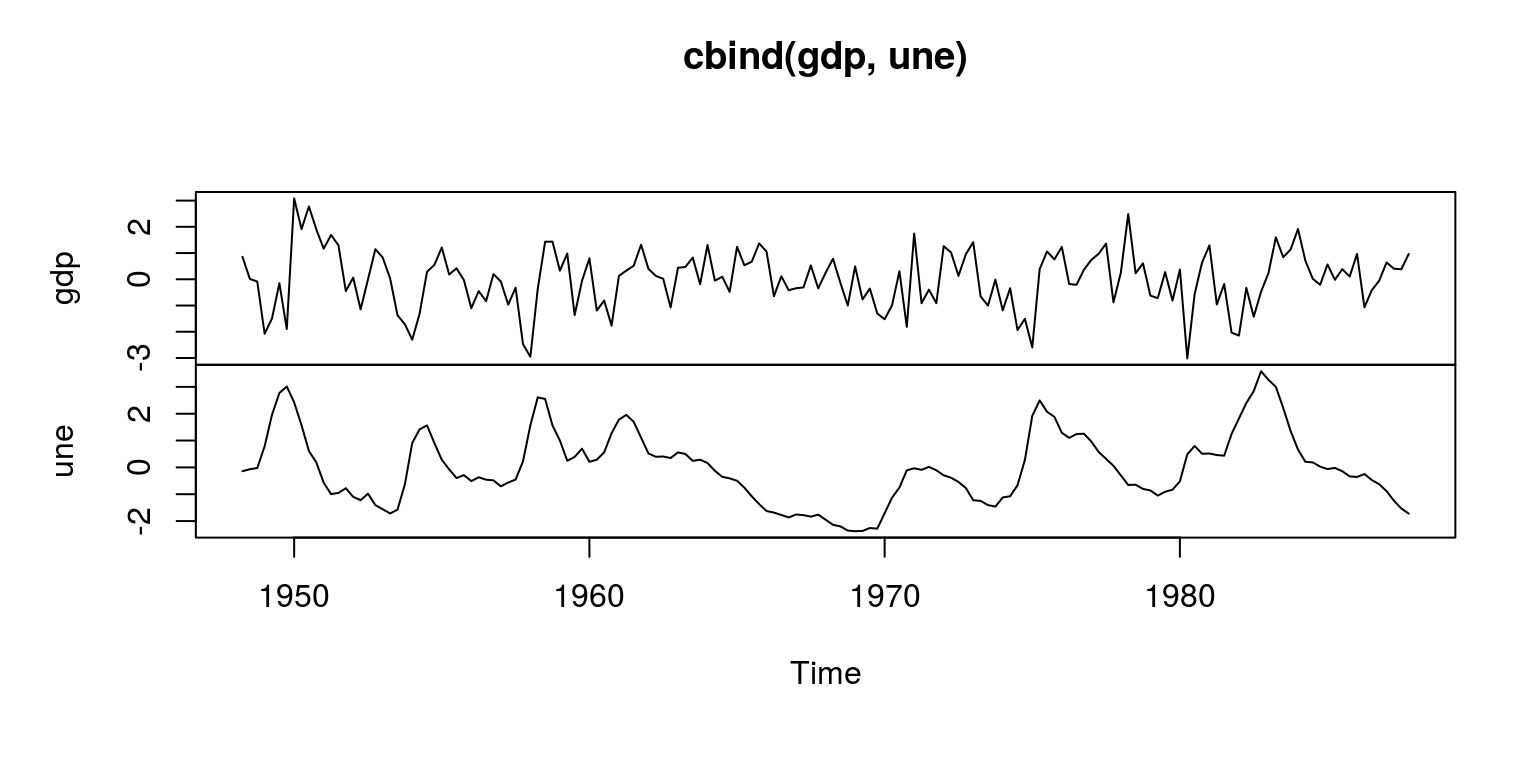To consider the degree of persistence in the data we make use of the autocorrelation function.

``gdp.acf <- ac(gdp, main = "output")``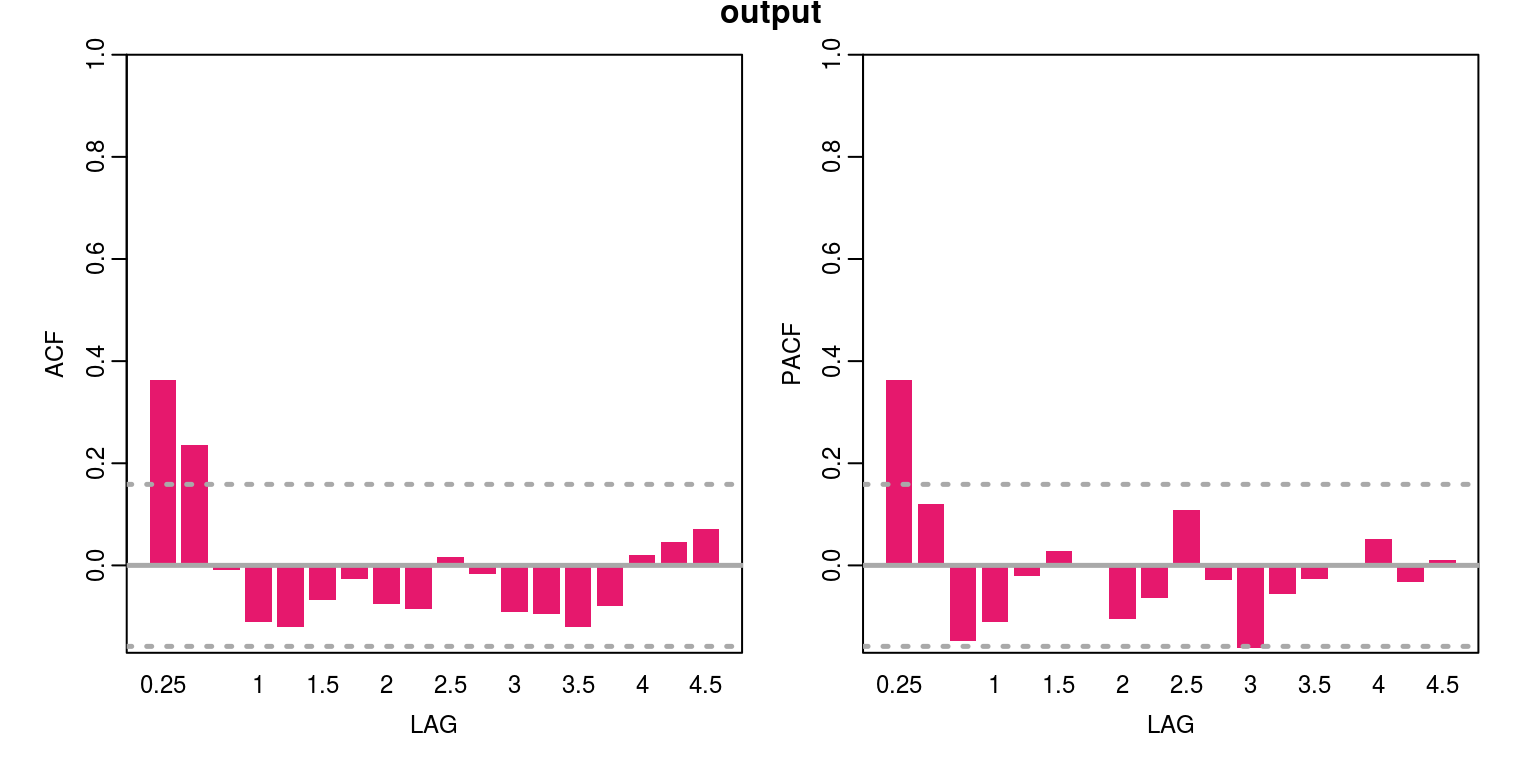``une.acf <- ac(une, main = "unemployent")``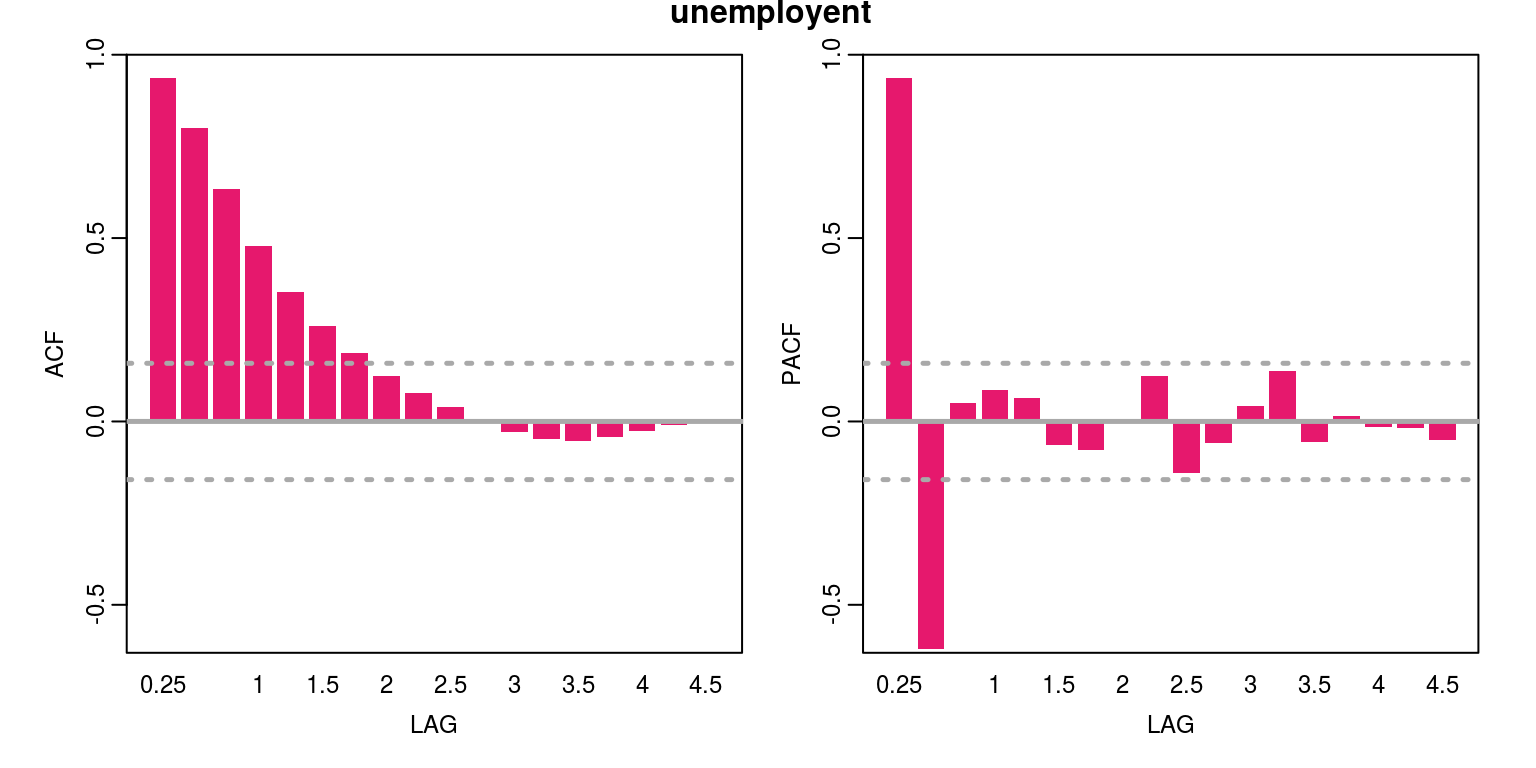As the persistence in the unemployment data is relatively high we need to check for a unit root, where we note that we able to reject null of unit root when using the Dickey-Fuller test.

``````adf.une <- ur.df(une, type = "trend", selectlags = "AIC")
``````##
## ###############################################
## # Augmented Dickey-Fuller Test Unit Root Test #
## ###############################################
##
## Test regression trend
##
##
## Call:
## lm(formula = z.diff ~ z.lag.1 + 1 + tt + z.diff.lag)
##
## Residuals:
##      Min       1Q   Median       3Q      Max
## -0.70448 -0.21077 -0.04731  0.19844  1.03067
##
## Coefficients:
##               Estimate Std. Error t value Pr(>|t|)
## (Intercept)  0.0059997  0.0536401   0.112    0.911
## z.lag.1     -0.0972035  0.0204701  -4.749 4.67e-06 ***
## tt          -0.0001167  0.0005838  -0.200    0.842
## z.diff.lag   0.6846610  0.0595395  11.499  < 2e-16 ***
## ---
## Signif. codes:
## 0 '***' 0.001 '**' 0.01 '*' 0.05 '.' 0.1 ' ' 1
##
## Residual standard error: 0.3311 on 153 degrees of freedom
## Multiple R-squared:  0.4776, Adjusted R-squared:  0.4674
## F-statistic: 46.63 on 3 and 153 DF,  p-value: < 2.2e-16
##
##
## Value of test-statistic is: -4.7486 7.5583 11.3199
##
## Critical values for test statistics:
##       1pct  5pct 10pct
## tau3 -3.99 -3.43 -3.13
## phi2  6.22  4.75  4.07
## phi3  8.43  6.49  5.47``````

## 1.1 Model selection and estimation

To create a bivariate object for the two time series we will model we can just column-bind the two existing objects. Thereafter, we can use information criteria to decide upon the number of lags to include.

``````dat.bv <- cbind(gdp, une)
colnames(dat.bv) <- c("gdp", "une")

info.bv <- VARselect(dat.bv, lag.max = 12, type = "const")
info.bv\$selection``````
``````## AIC(n)  HQ(n)  SC(n) FPE(n)
##      2      2      2      2``````

Note that all of the information criteria suggest the use of 2 lags would be appropriate, which would imply that we need to set `p=2` when estimating the model, as we do below:

``````bv.est <- VAR(dat.bv, p = 2, type = "const", season = NULL,
exog = NULL)
summary(bv.est)``````
``````##
## VAR Estimation Results:
## =========================
## Endogenous variables: gdp, une
## Deterministic variables: const
## Sample size: 157
## Log Likelihood: -211.593
## Roots of the characteristic polynomial:
## 0.8361 0.8361 0.1014 0.1014
## Call:
## VAR(y = dat.bv, p = 2, type = "const", exogen = NULL)
##
##
## Estimation results for equation gdp:
## ====================================
## gdp = gdp.l1 + une.l1 + gdp.l2 + une.l2 + const
##
##        Estimate Std. Error t value Pr(>|t|)
## gdp.l1  0.06905    0.10782   0.640  0.52289
## une.l1 -0.61530    0.30723  -2.003  0.04699 *
## gdp.l2  0.04741    0.09098   0.521  0.60309
## une.l2  0.90193    0.31426   2.870  0.00469 **
## const  -0.01570    0.07684  -0.204  0.83833
## ---
## Signif. codes:
## 0 '***' 0.001 '**' 0.01 '*' 0.05 '.' 0.1 ' ' 1
##
##
## Residual standard error: 0.9613 on 152 degrees of freedom
## Multiple R-Squared: 0.2687,  Adjusted R-squared: 0.2495
## F-statistic: 13.97 on 4 and 152 DF,  p-value: 1e-09
##
##
## Estimation results for equation une:
## ====================================
## une = gdp.l1 + une.l1 + gdp.l2 + une.l2 + const
##
##         Estimate Std. Error t value Pr(>|t|)
## gdp.l1 -0.119156   0.035975  -3.312 0.001157 **
## une.l1  1.333482   0.102510  13.008  < 2e-16 ***
## gdp.l2 -0.029883   0.030358  -0.984 0.326497
## une.l2 -0.416951   0.104853  -3.977 0.000108 ***
## const  -0.007501   0.025639  -0.293 0.770243
## ---
## Signif. codes:
## 0 '***' 0.001 '**' 0.01 '*' 0.05 '.' 0.1 ' ' 1
##
##
## Residual standard error: 0.3207 on 152 degrees of freedom
## Multiple R-Squared: 0.9429,  Adjusted R-squared: 0.9414
## F-statistic: 628.1 on 4 and 152 DF,  p-value: < 2.2e-16
##
##
##
## Covariance matrix of residuals:
##         gdp     une
## gdp  0.9240 -0.2022
## une -0.2022  0.1029
##
## Correlation matrix of residuals:
##         gdp     une
## gdp  1.0000 -0.6558
## une -0.6558  1.0000``````

Note that the results suggest that the system is stable (the characteristic roots may be interpreted as eigenvalues in this case). Note also that there would appear to be many insignificant variables in this model, where output is influenced by past unemployment; and unemployment is influenced by past measures of output and unemployment.

To consider the model fit we can perform some diagnostic tests on residuals of the model. To test for serial correlation we can apply a Portmanteau-test, which is implemented as follows:

``````bv.serial <- serial.test(bv.est, lags.pt = 12, type = "PT.asymptotic")
bv.serial``````
``````##
##  Portmanteau Test (asymptotic)
##
## data:  Residuals of VAR object bv.est
## Chi-squared = 46.579, df = 40, p-value = 0.22``````
``plot(bv.serial, names = "gdp")``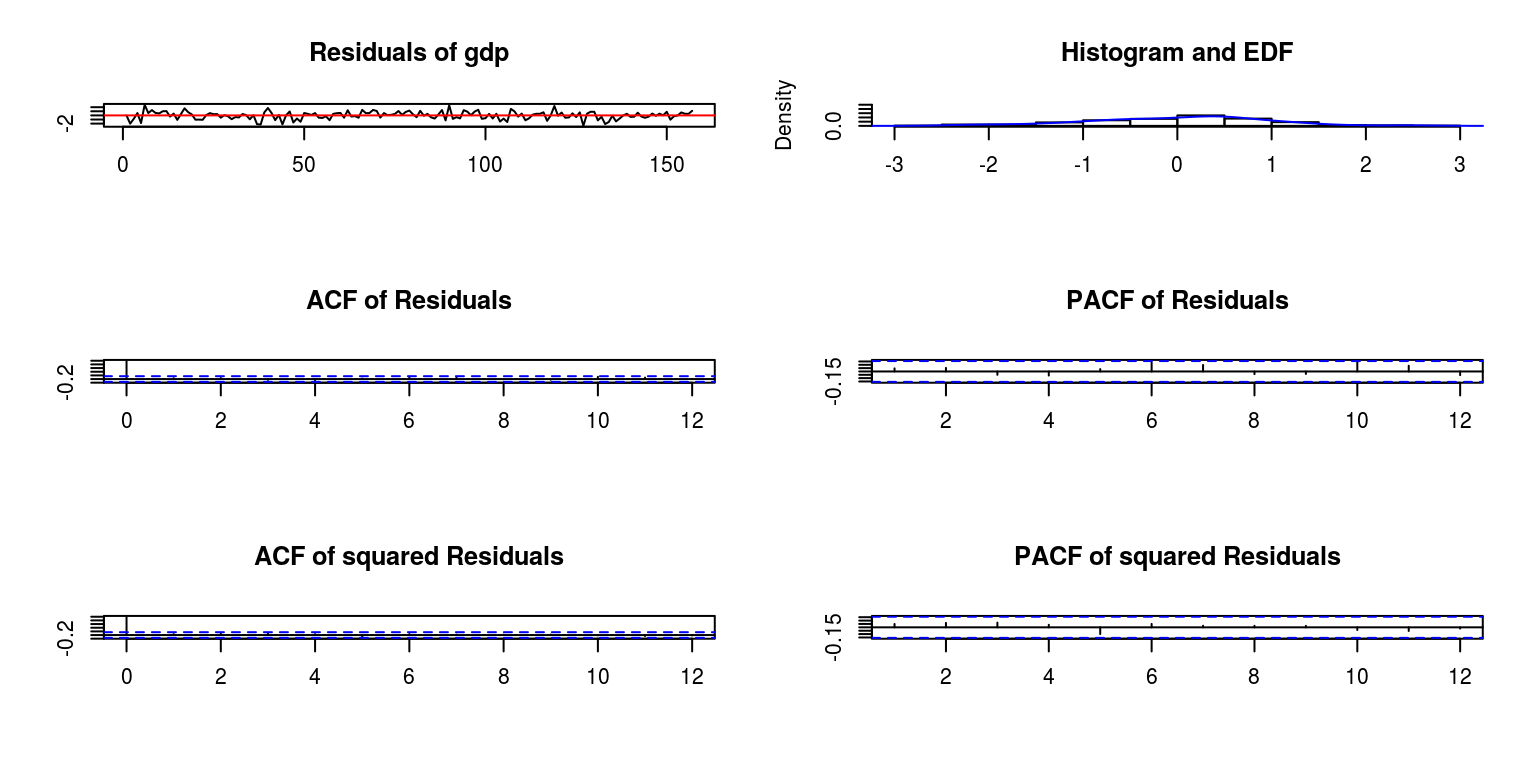``plot(bv.serial, names = "une")``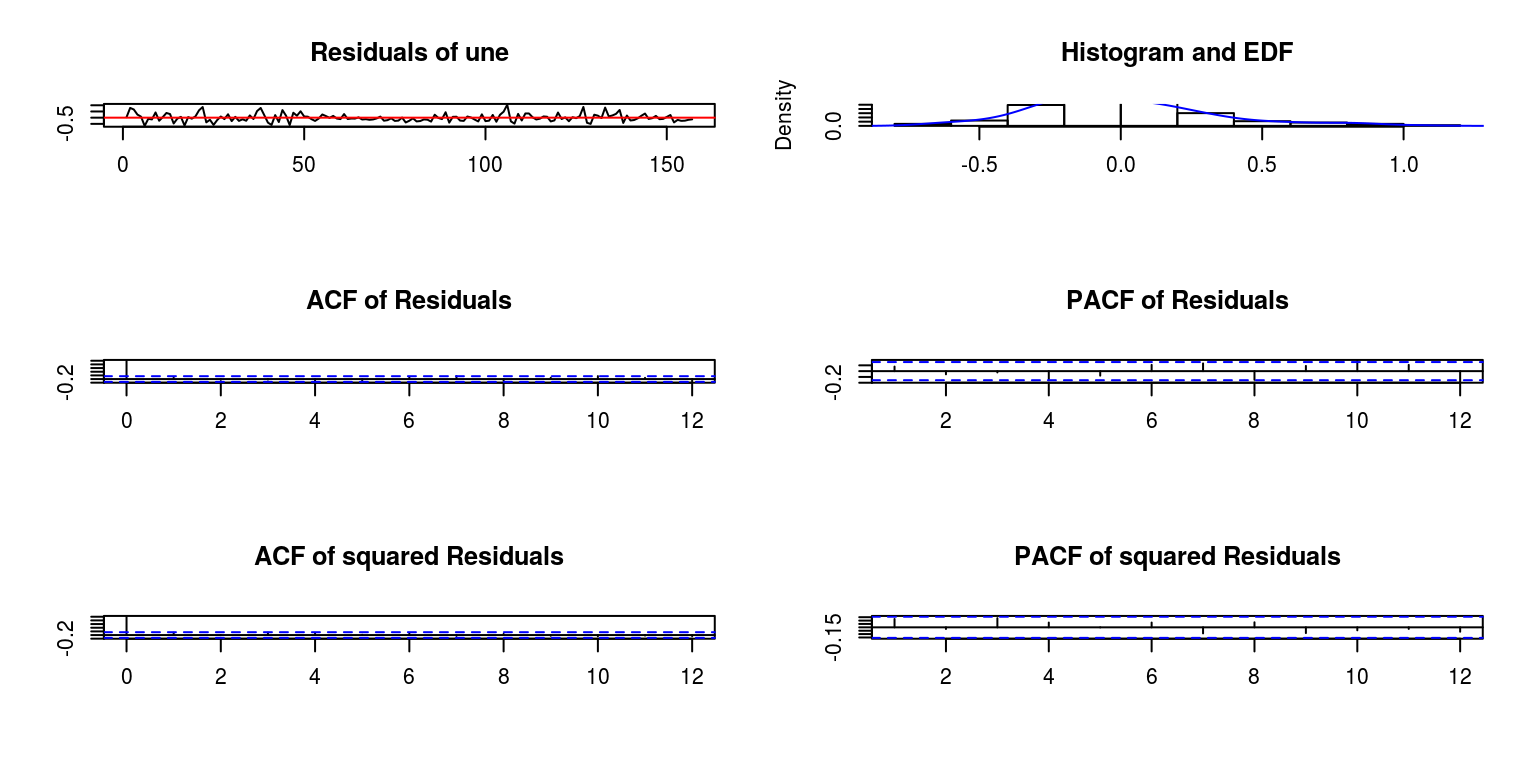To interpret these statistics note that a p-value that is greater than 5% would generally indicate that there is an absence of serial correlation. To test for heteroscedasticity in the residuals we can perform a multivariate ARCH Lagrange-Multiplier test.

``````bv.arch <- arch.test(bv.est, lags.multi = 12, multivariate.only = TRUE)
bv.arch``````
``````##
##  ARCH (multivariate)
##
## data:  Residuals of VAR object bv.est
## Chi-squared = 108.4, df = 108, p-value = 0.4712``````

Once again the p-value that is greater than 5% would indicate the absence of heteroscedasticity. To consider the distribution of the residuals, we could apply a normality test.

``````bv.norm <- normality.test(bv.est, multivariate.only = TRUE)
bv.norm``````
``````## \$JB
##
##  JB-Test (multivariate)
##
## data:  Residuals of VAR object bv.est
## Chi-squared = 2.3194, df = 4, p-value = 0.6772
##
##
## \$Skewness
##
##  Skewness only (multivariate)
##
## data:  Residuals of VAR object bv.est
## Chi-squared = 2.1157, df = 2, p-value = 0.3472
##
##
## \$Kurtosis
##
##  Kurtosis only (multivariate)
##
## data:  Residuals of VAR object bv.est
## Chi-squared = 0.20376, df = 2, p-value = 0.9031``````

where the resulting p-value indicates that the residuals are fairly normally distributed. Then lastly to test for the structural break in the residuals we can apply a CUSUM test.

``````bv.cusum <- stability(bv.est, type = "OLS-CUSUM")
plot(bv.cusum)``````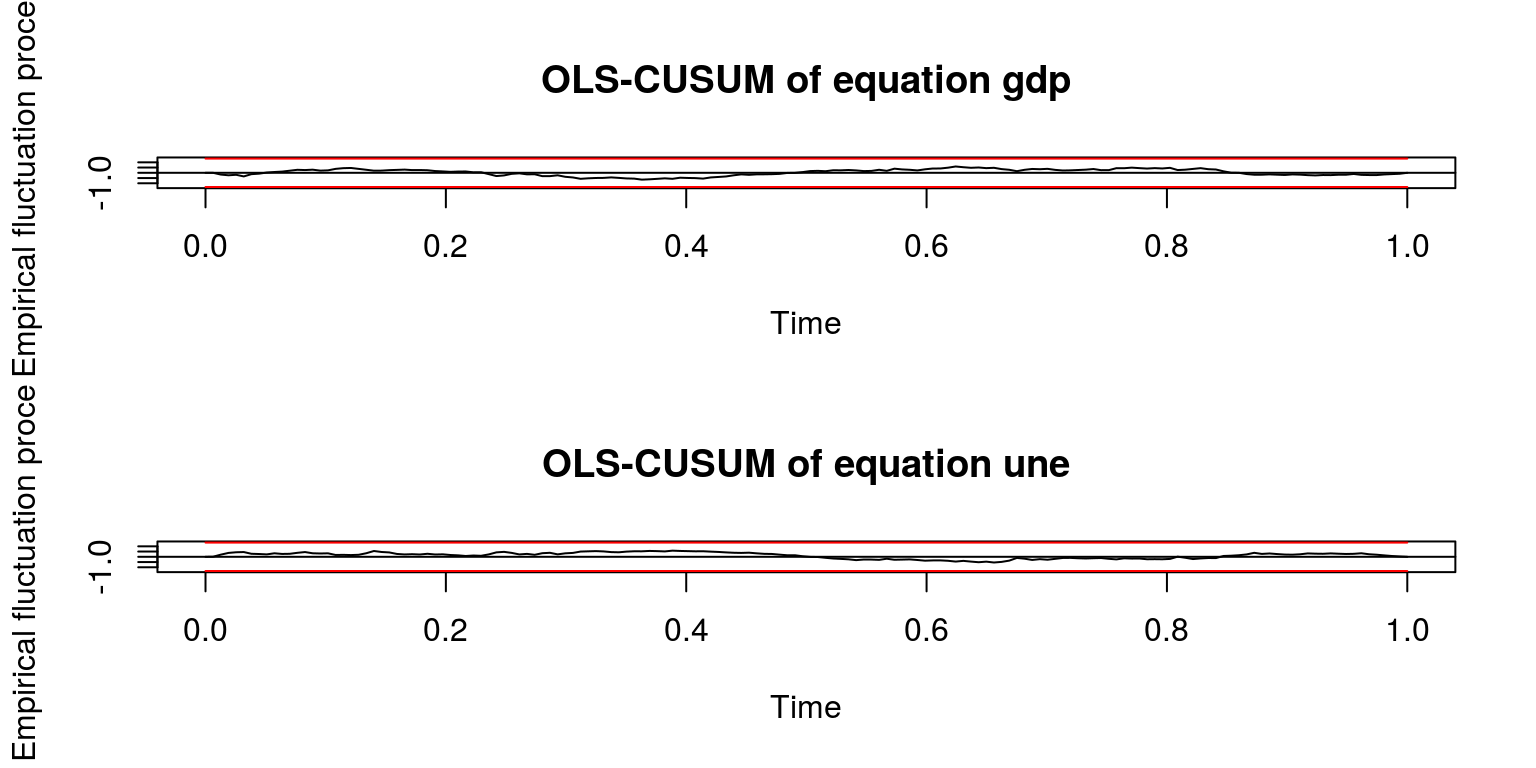where there does not appear to be a break in the respective confidence intervals.

## 1.2 Granger causality, IRFs and variance decompositions

We are then able to test for Granger causality, where we note that the null hypothesis of no Granger causality is dismissed in both directions.

``````bv.cause.gdp <- causality(bv.est, cause = "gdp")
bv.cause.gdp``````
``````## \$Granger
##
##  Granger causality H0: gdp do not Granger-cause
##  une
##
## data:  VAR object bv.est
## F-Test = 5.5723, df1 = 2, df2 = 304, p-value =
## 0.0042
##
##
## \$Instant
##
##  H0: No instantaneous causality between: gdp and
##  une
##
## data:  VAR object bv.est
## Chi-squared = 47.214, df = 1, p-value =
## 6.364e-12``````
``````bv.cause.une <- causality(bv.est, cause = "une")
bv.cause.une``````
``````## \$Granger
##
##  Granger causality H0: une do not Granger-cause
##  gdp
##
## data:  VAR object bv.est
## F-Test = 12.821, df1 = 2, df2 = 304, p-value =
## 4.511e-06
##
##
## \$Instant
##
##  H0: No instantaneous causality between: une and
##  gdp
##
## data:  VAR object bv.est
## Chi-squared = 47.214, df = 1, p-value =
## 6.364e-12``````

To generate impulse response functions to describe the reponse of output to an unemployment shock, we proceed as follows:

``````irf.gdp <- irf(bv.est, impulse = "une", response = "gdp",
n.ahead = 40, boot = TRUE)
plot(irf.gdp, ylab = "ouput", main = "Shock from unemployment")``````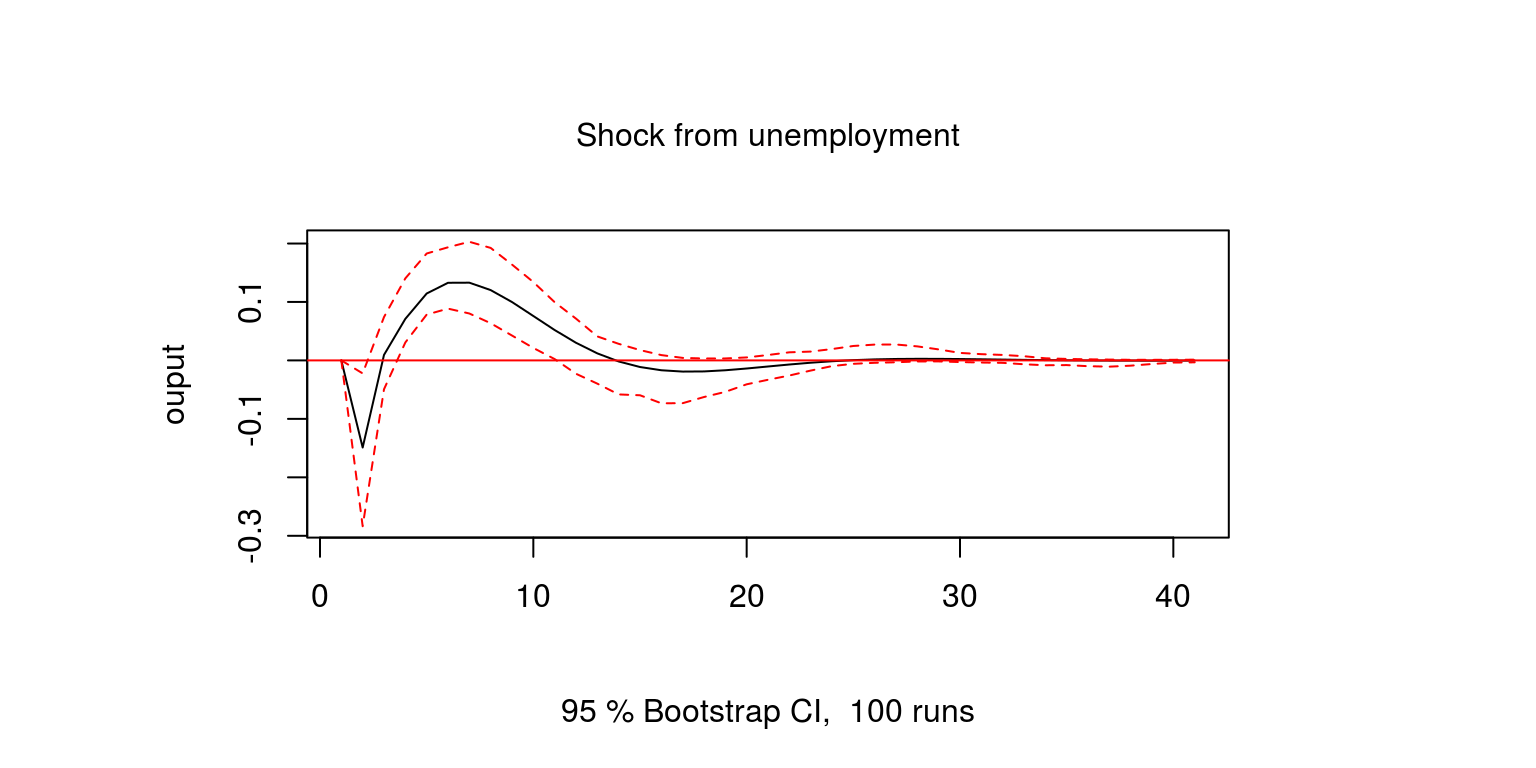Note that a positive shock to unemployment has a negative affect on ouput (i.e. lower spending power). To consider the reponse of unemployment to output shock we execute the command.

``````irf.une <- irf(bv.est, impulse = "gdp", response = "une",
n.ahead = 40, boot = TRUE)
plot(irf.une, ylab = "unemployment", main = "Shock from output")``````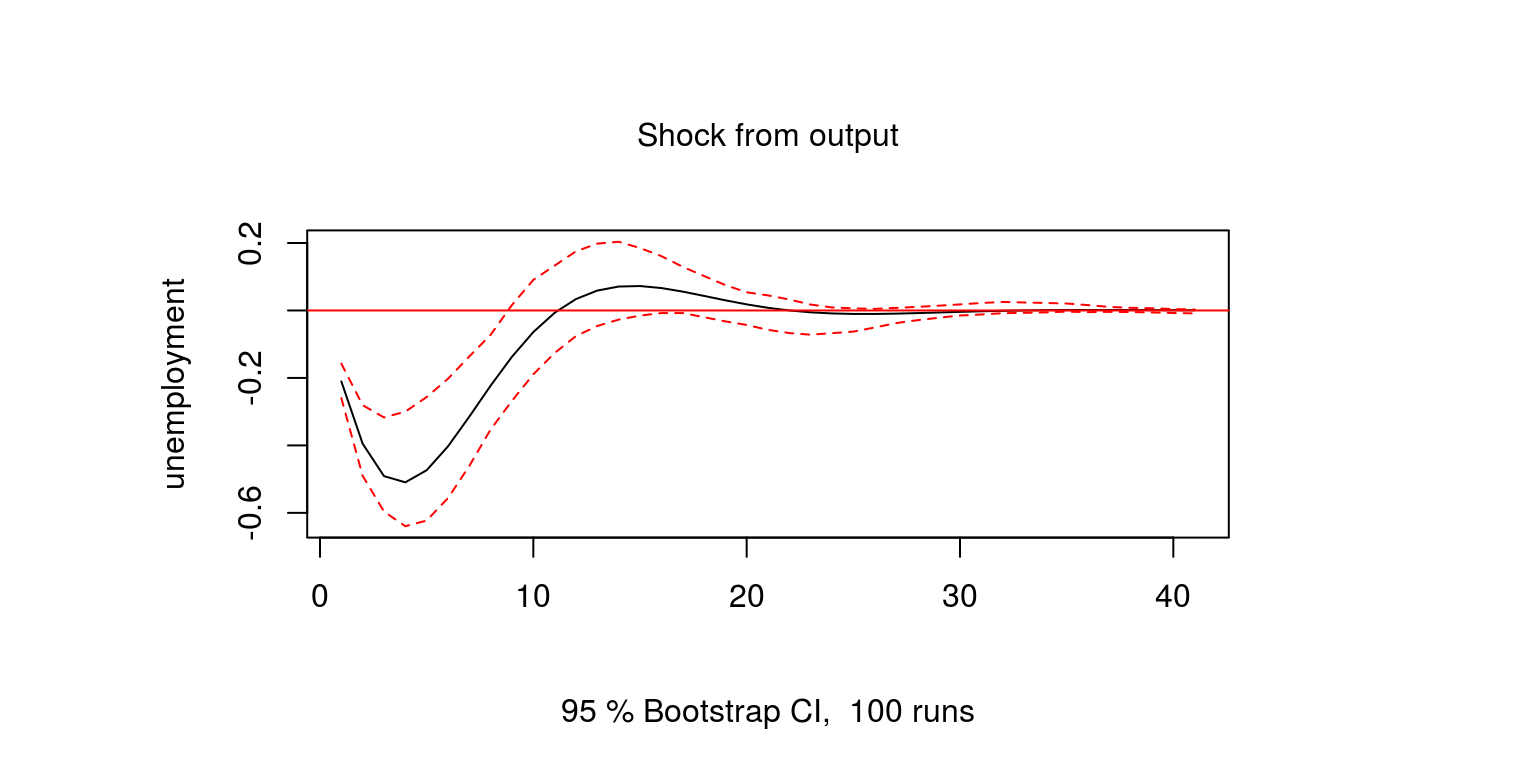Here we note that a positive shock to output decreases unemployment by a relatively large and persistent amount. Similarly, we can look at the effect of an unemployment shock on unemployment.

``````irf.une_un <- irf(bv.est, impulse = "une", response = "une",
n.ahead = 40, boot = TRUE)
plot(irf.une_un, ylab = "unemployment", main = "Shock from unemployment")``````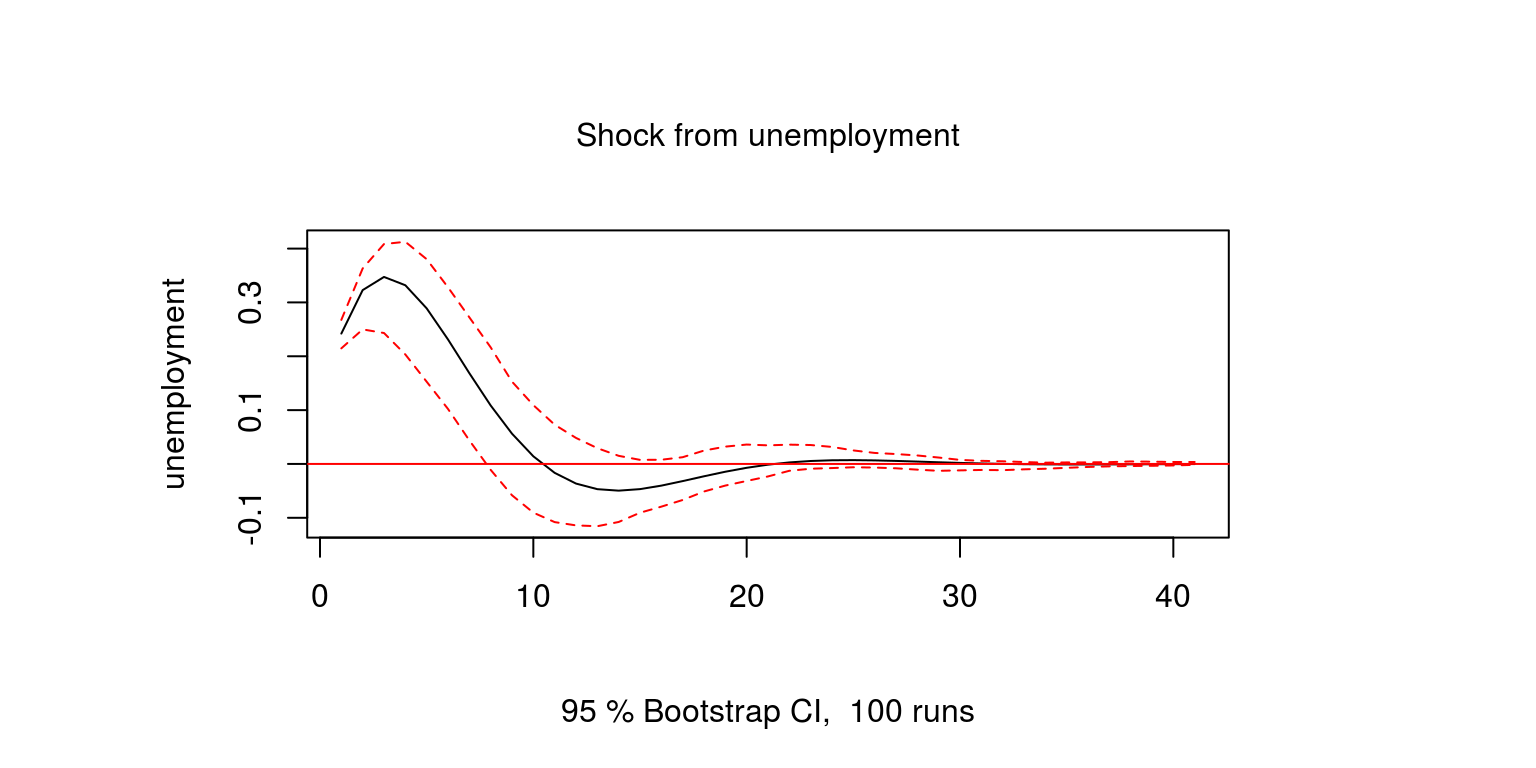To generate the forecast error variance decompositions we make use of the `fevd` command, where we set the number of steps ahead to ten.

``````bv.vardec <- fevd(bv.est, n.ahead = 10)
plot(bv.vardec)``````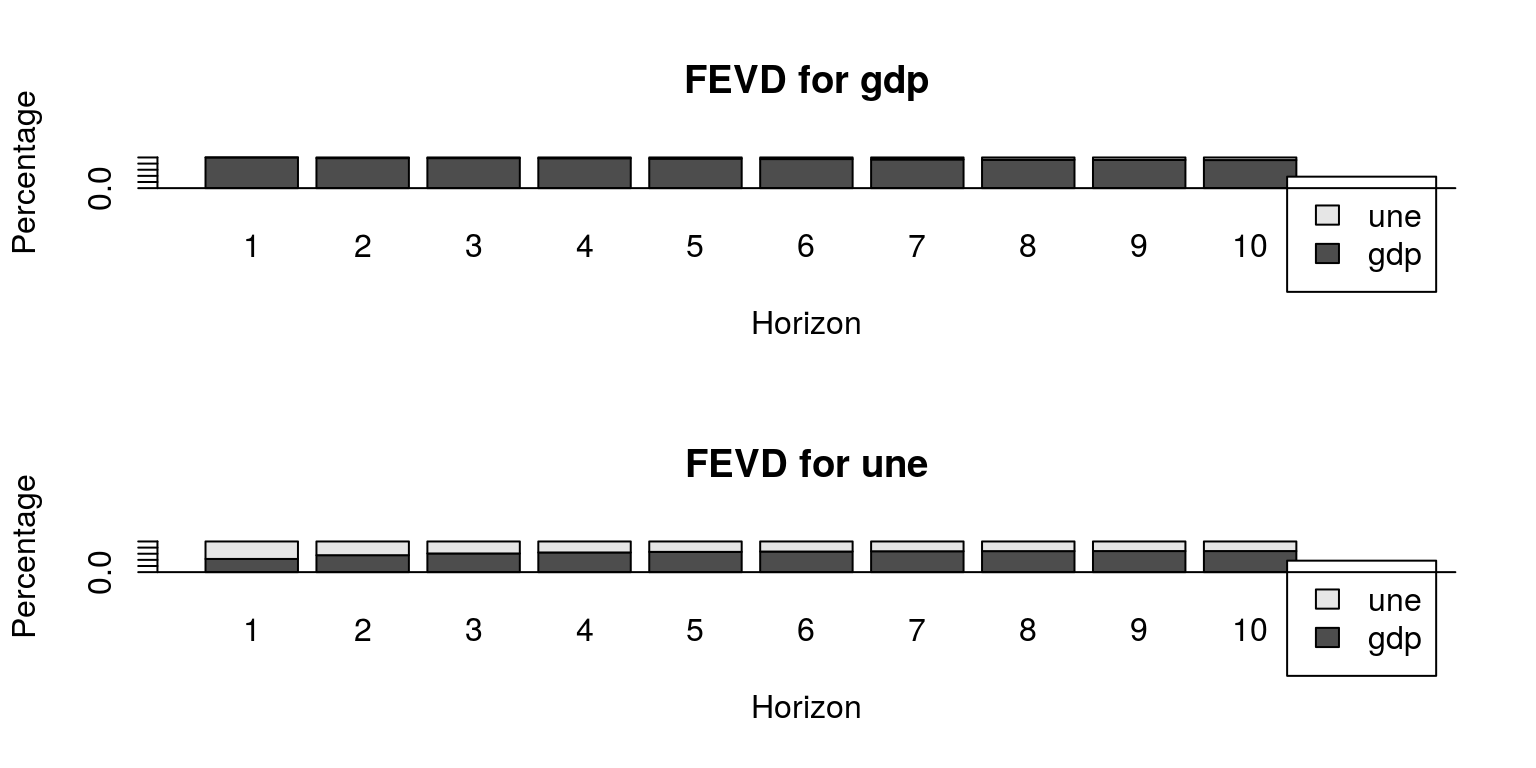Note that these results suggest that output is largely determined by output shocks, while unemployment is influenced by both shocks.

## 1.3 Forecasting

To forecast forward we can make use of the `predict` command, where in this case we are forecasting 8 steps ahead. We are also looking to make use of 95% confidence intervals for the forecast.

``````predictions <- predict(bv.est, n.ahead = 8, ci = 0.95)
plot(predictions, names = "gdp")``````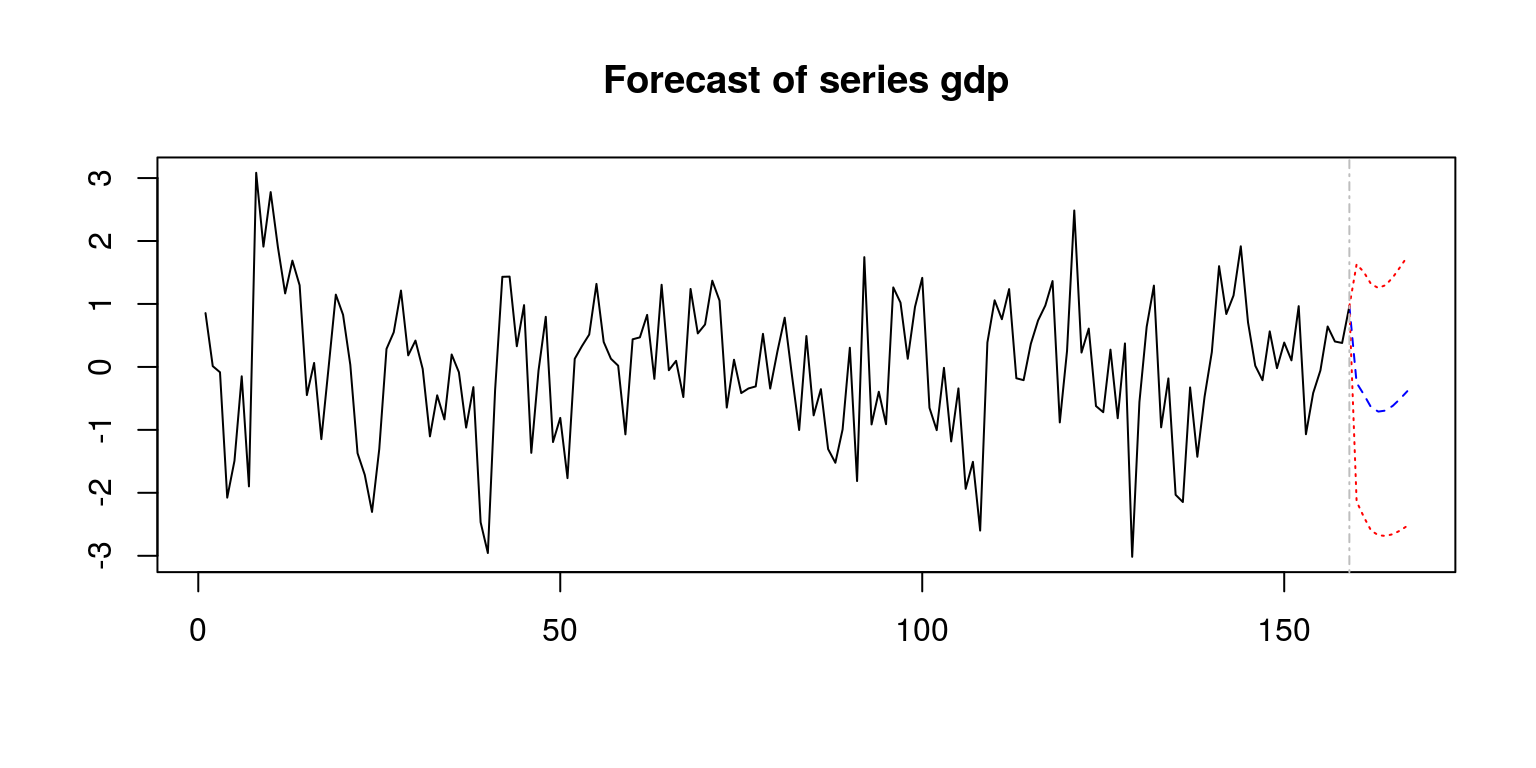``plot(predictions, names = "une")``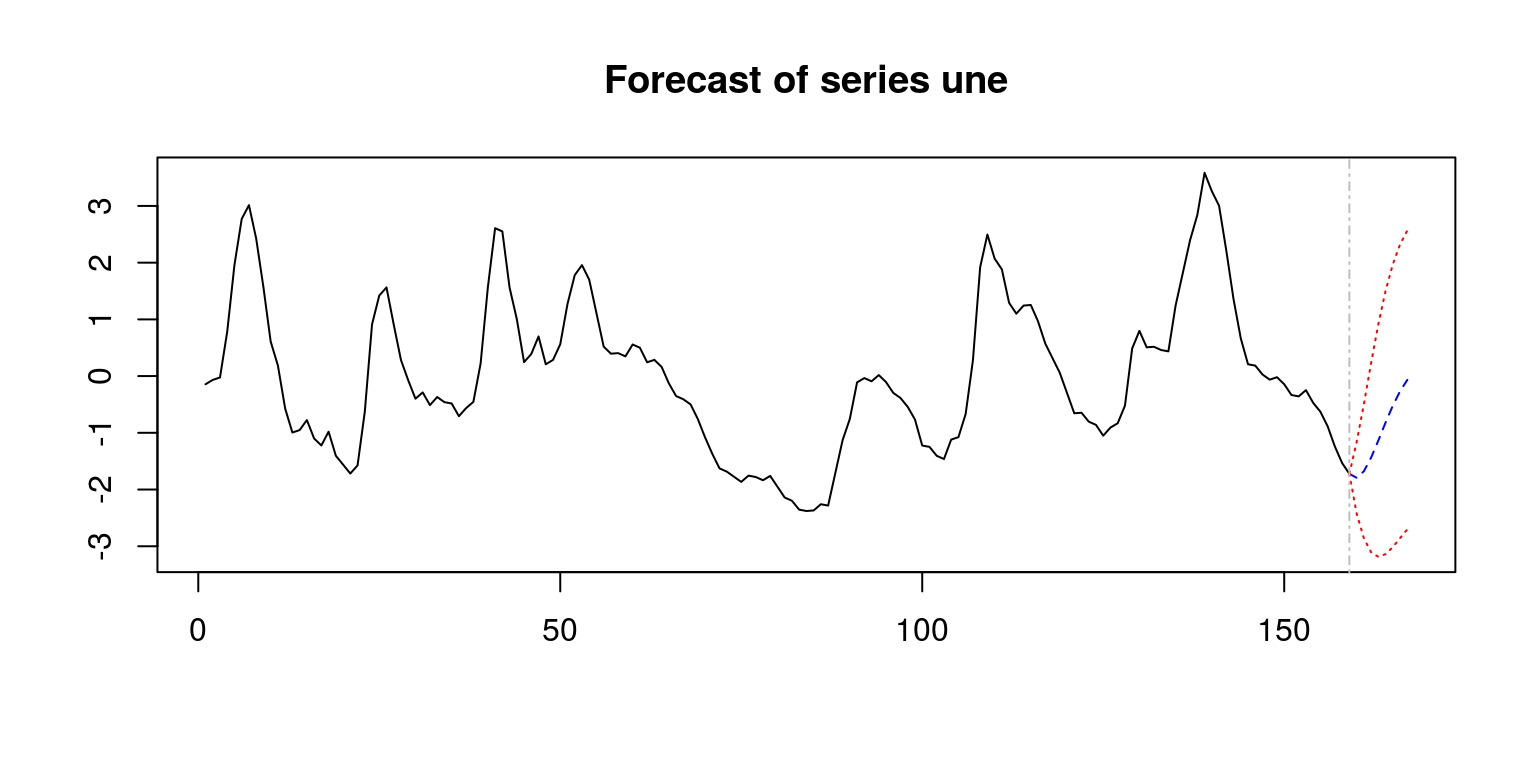These could also be displayed with the aid of fancharts for the forecasts.

``fanchart(predictions, names = "gdp")``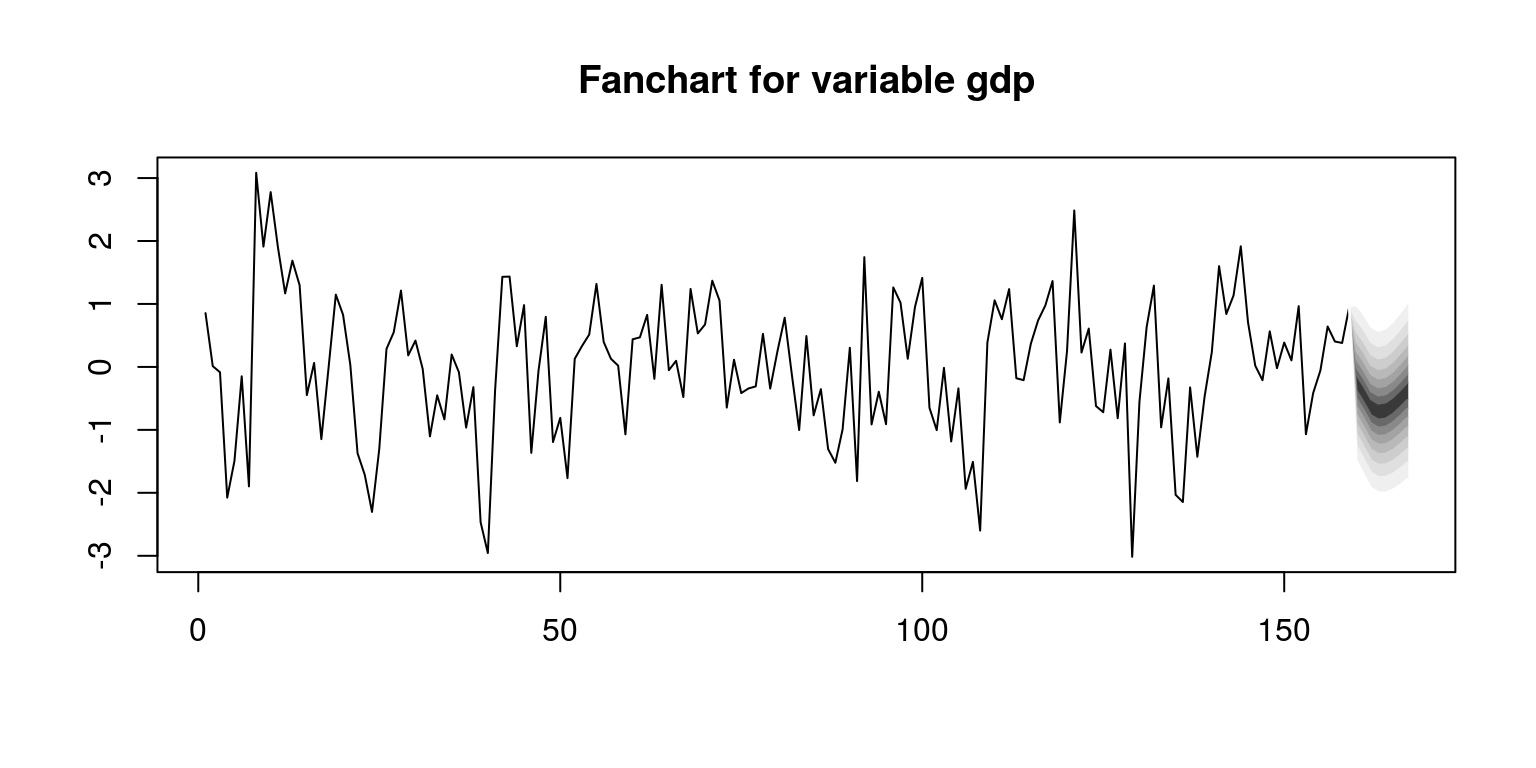``fanchart(predictions, names = "une")``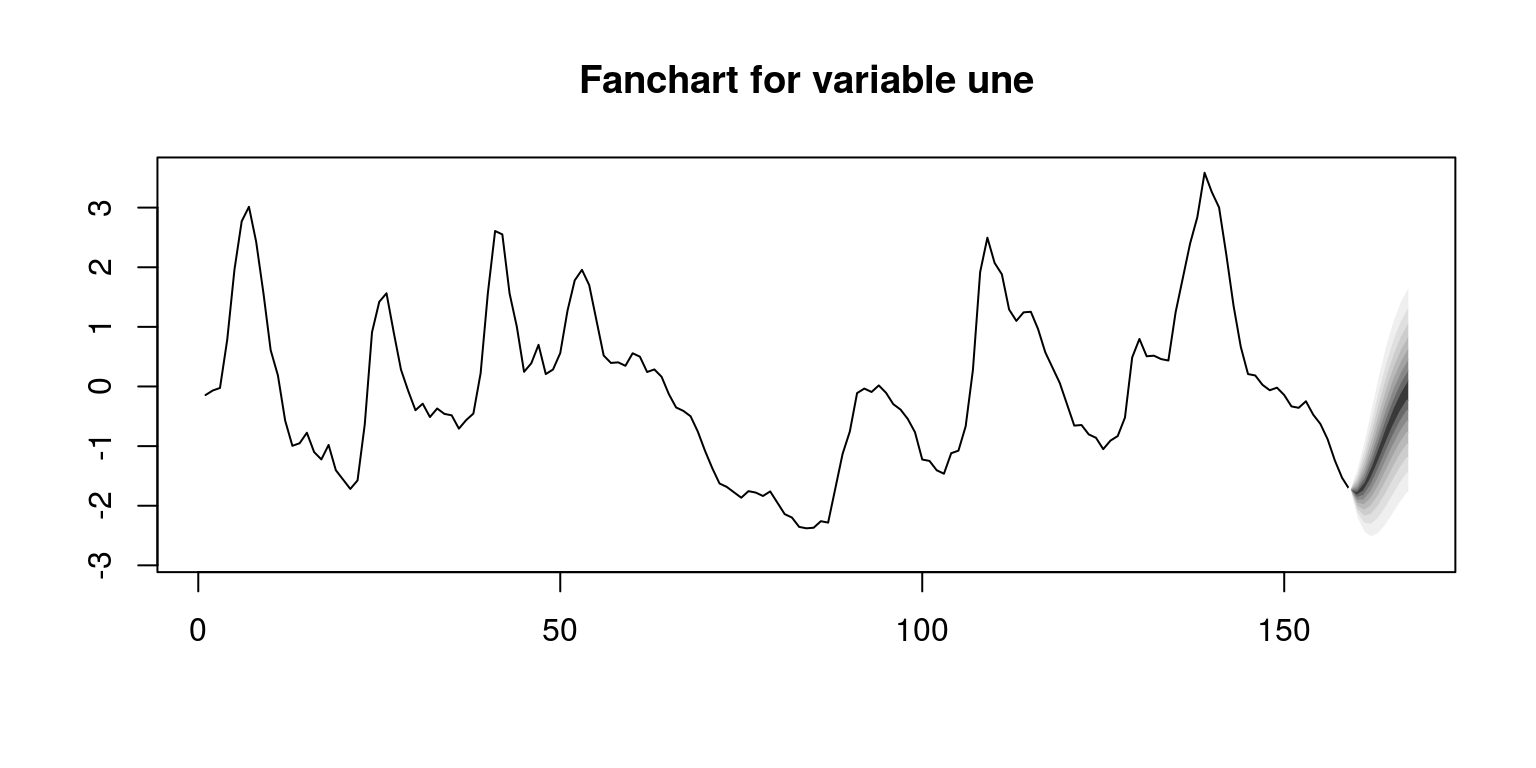# 2 VAR model for key South African macroeconomic data

The next exercise makes use of measures of South African output, inflation and interest rates. This example is contained in the file `T7-var.R`, where we could proceed as we did previosuly.

``````rm(list = ls())
graphics.off()

library(tsm)
library(vars)
library(mFilter)

setwd("C:\\Users\\image")``````

Note that we have included the `mFilter` package as we want to use the Hodrick-Prescott filter to derive an estimate for the South African output gap. To load the data and create the respective time series objects, we proceed as follows:

``````dat_sa <- read.csv(file = "data_sa.csv")

gdp <- ts(log(dat_sa\$gdp), start = c(1981, 2), freq = 4)
inf <- ts(dat_sa\$inf, start = c(1981, 2), freq = 4)
int <- ts(dat_sa\$int, start = c(1981, 2), freq = 4)

plot(cbind(gdp, inf, int))``````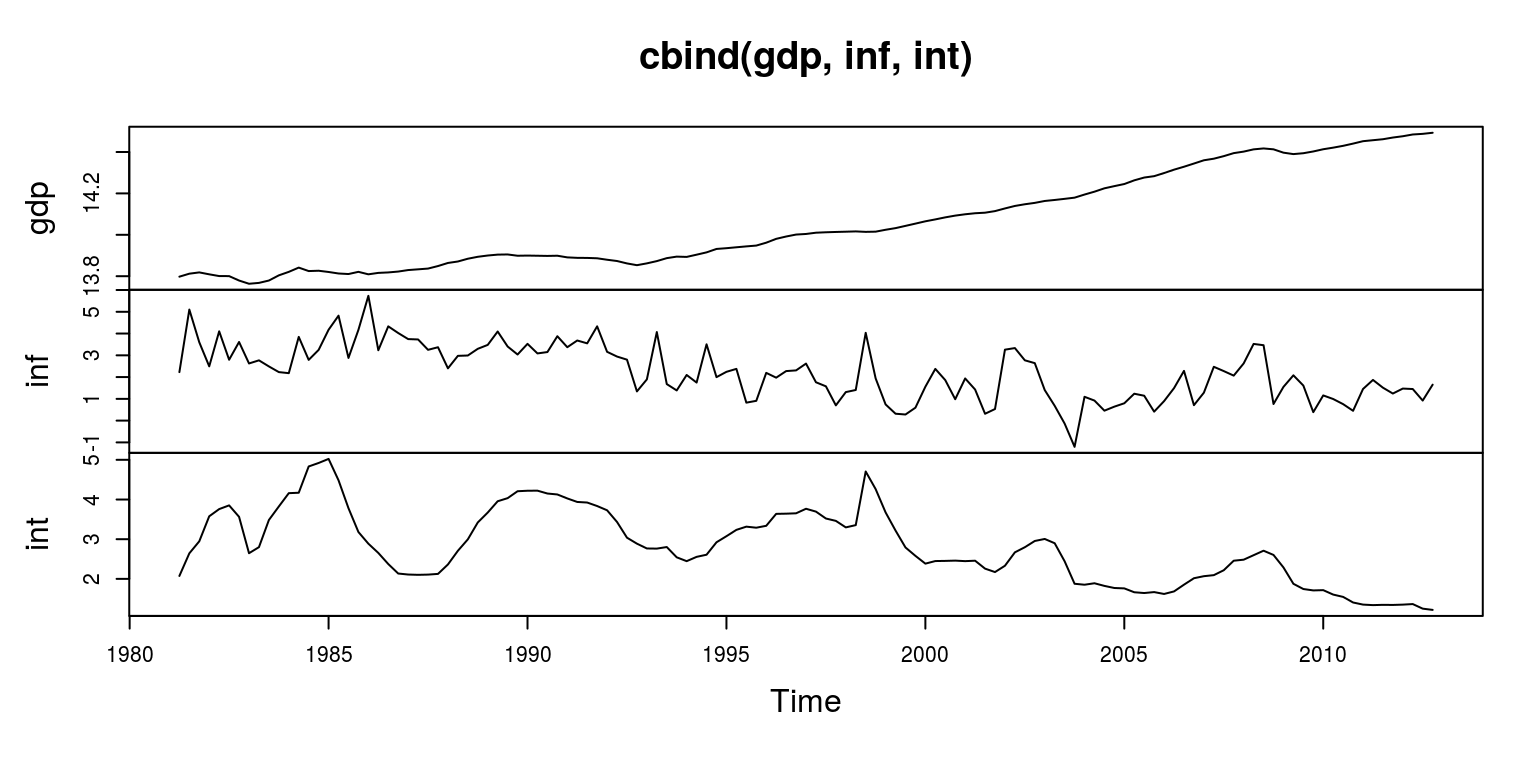As noted, the Hodrick-Prescott filter is used to derive a measure of the output gap, where the smoothing parameter is set at `1600`.

``````gdp.hp <- hpfilter(gdp, freq = 1600)
gdp.gap <- gdp.hp\$cycle``````

To consider the degree of persistence in the data we make use of the autocorrelation function.

``gdp.acf <- ac(gdp.gap, main = "GDP")``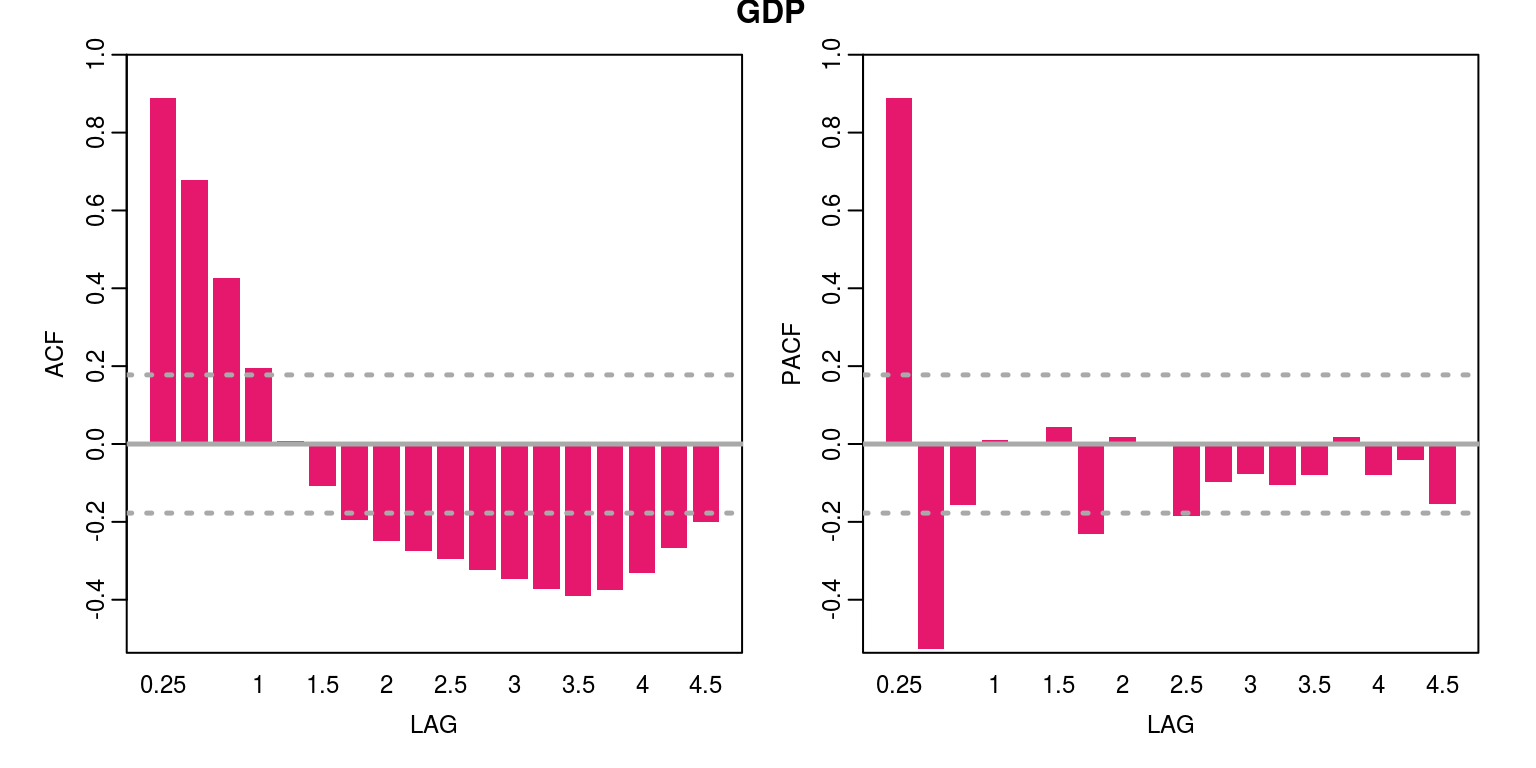``inf.acf <- ac(inf, main = "INF")``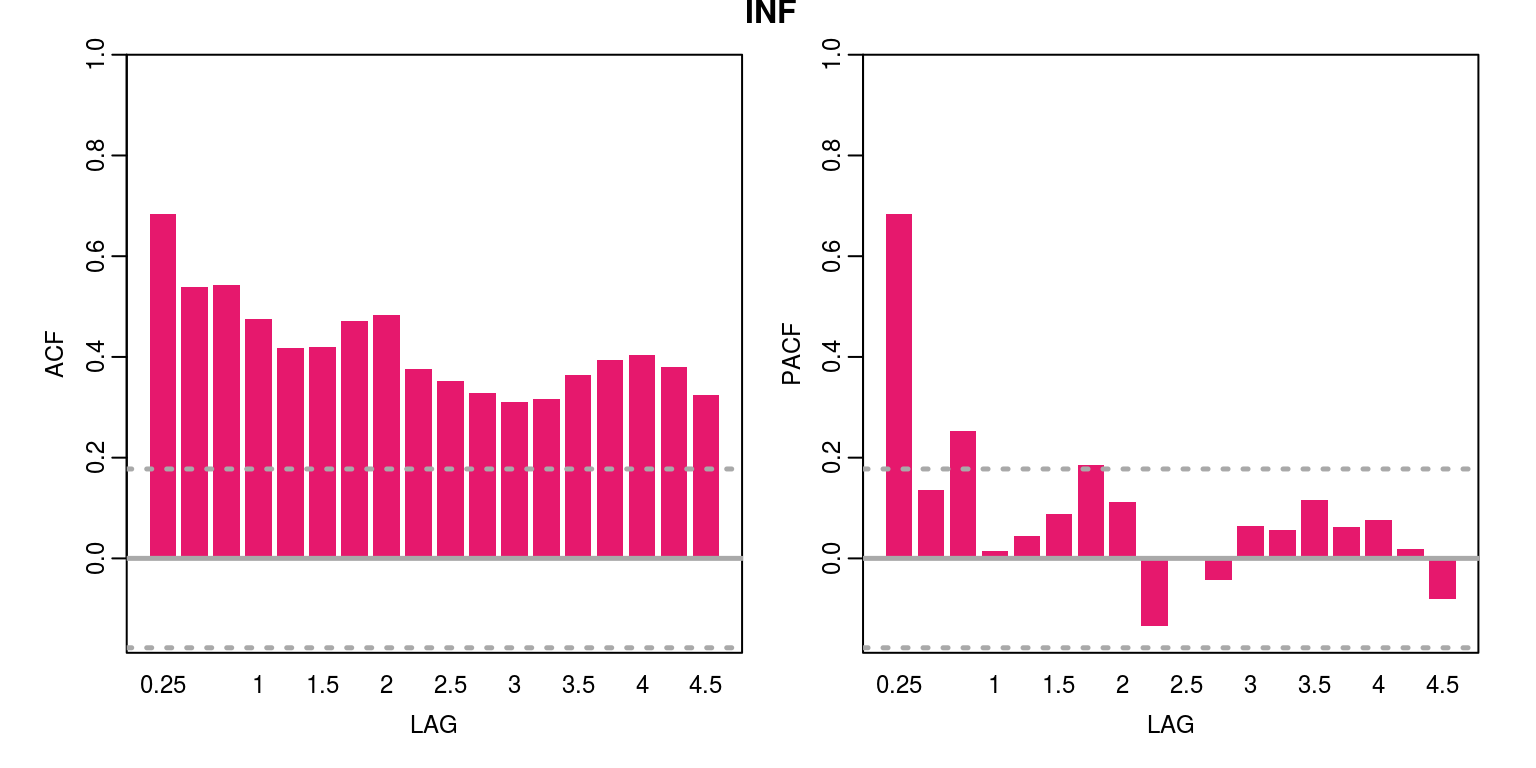``int.acf <- ac(int, main = "INT")``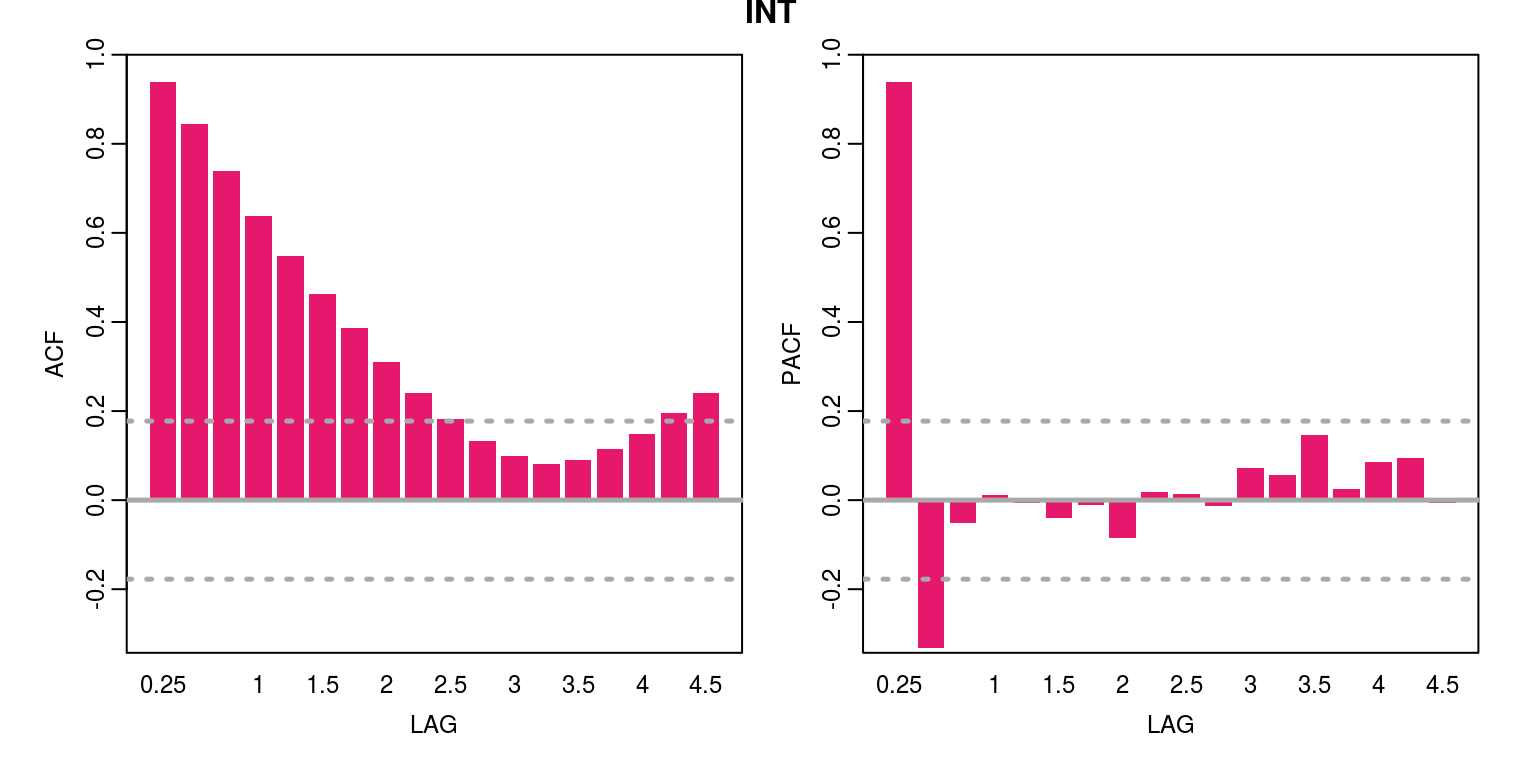As the persistence in the data is relatively high we need to check for a unit root, where we note that we able to reject null of unit root when using the Dickey-Fuller test for each variable.

``````adf.gdp <- ur.df(gdp.gap, type = "trend", selectlags = "AIC")
``````##
## ###############################################
## # Augmented Dickey-Fuller Test Unit Root Test #
## ###############################################
##
## Test regression trend
##
##
## Call:
## lm(formula = z.diff ~ z.lag.1 + 1 + tt + z.diff.lag)
##
## Residuals:
##        Min         1Q     Median         3Q        Max
## -0.0225345 -0.0024890  0.0003481  0.0032606  0.0144515
##
## Coefficients:
##               Estimate Std. Error t value Pr(>|t|)
## (Intercept) -5.408e-04  1.089e-03  -0.497    0.620
## z.lag.1     -1.718e-01  3.585e-02  -4.793 4.72e-06 ***
## tt           6.277e-06  1.482e-05   0.423    0.673
## z.diff.lag   5.179e-01  7.590e-02   6.824 3.75e-10 ***
## ---
## Signif. codes:
## 0 '***' 0.001 '**' 0.01 '*' 0.05 '.' 0.1 ' ' 1
##
## Residual standard error: 0.00598 on 121 degrees of freedom
## Multiple R-squared:  0.3222, Adjusted R-squared:  0.3054
## F-statistic: 19.18 on 3 and 121 DF,  p-value: 3.071e-10
##
##
## Value of test-statistic is: -4.7928 7.7343 11.5687
##
## Critical values for test statistics:
##       1pct  5pct 10pct
## tau3 -3.99 -3.43 -3.13
## phi2  6.22  4.75  4.07
## phi3  8.43  6.49  5.47``````
``````adf.inf <- ur.df(inf, type = "trend", selectlags = "AIC")
``````##
## ###############################################
## # Augmented Dickey-Fuller Test Unit Root Test #
## ###############################################
##
## Test regression trend
##
##
## Call:
## lm(formula = z.diff ~ z.lag.1 + 1 + tt + z.diff.lag)
##
## Residuals:
##     Min      1Q  Median      3Q     Max
## -1.9945 -0.6089  0.0336  0.4645  2.2854
##
## Coefficients:
##              Estimate Std. Error t value Pr(>|t|)
## (Intercept)  1.815286   0.375612   4.833 3.99e-06 ***
## z.lag.1     -0.512318   0.092779  -5.522 1.95e-07 ***
## tt          -0.010878   0.002964  -3.669 0.000363 ***
## z.diff.lag  -0.025617   0.088079  -0.291 0.771666
## ---
## Signif. codes:
## 0 '***' 0.001 '**' 0.01 '*' 0.05 '.' 0.1 ' ' 1
##
## Residual standard error: 0.8422 on 121 degrees of freedom
## Multiple R-squared:  0.2678, Adjusted R-squared:  0.2496
## F-statistic: 14.75 on 3 and 121 DF,  p-value: 3.024e-08
##
##
## Value of test-statistic is: -5.5219 10.2606 15.3089
##
## Critical values for test statistics:
##       1pct  5pct 10pct
## tau3 -3.99 -3.43 -3.13
## phi2  6.22  4.75  4.07
## phi3  8.43  6.49  5.47``````
``````adf.int <- ur.df(int, type = "trend", selectlags = "AIC")
``````##
## ###############################################
## # Augmented Dickey-Fuller Test Unit Root Test #
## ###############################################
##
## Test regression trend
##
##
## Call:
## lm(formula = z.diff ~ z.lag.1 + 1 + tt + z.diff.lag)
##
## Residuals:
##      Min       1Q   Median       3Q      Max
## -0.82058 -0.09310 -0.01033  0.09363  1.41182
##
## Coefficients:
##               Estimate Std. Error t value Pr(>|t|)
## (Intercept)  0.5067585  0.1336958   3.790 0.000236 ***
## z.lag.1     -0.1265458  0.0322572  -3.923 0.000146 ***
## tt          -0.0024535  0.0008079  -3.037 0.002929 **
## z.diff.lag   0.4696463  0.0769221   6.105 1.28e-08 ***
## ---
## Signif. codes:
## 0 '***' 0.001 '**' 0.01 '*' 0.05 '.' 0.1 ' ' 1
##
## Residual standard error: 0.2378 on 121 degrees of freedom
## Multiple R-squared:  0.2842, Adjusted R-squared:  0.2665
## F-statistic: 16.02 on 3 and 121 DF,  p-value: 7.847e-09
##
##
## Value of test-statistic is: -3.923 5.2709 7.8253
##
## Critical values for test statistics:
##       1pct  5pct 10pct
## tau3 -3.99 -3.43 -3.13
## phi2  6.22  4.75  4.07
## phi3  8.43  6.49  5.47``````

## 2.1 Model selection and estimation

To investigate the relationship between the interest rate and the inflation rate, we create a bivariate object for the two time series. Thereafter, we can use information criteria to decide upon the number of lags to include.

``````dat.bv <- cbind(int, inf)
colnames(dat.bv) <- c("int", "inf")

info.bv <- VARselect(dat.bv, lag.max = 12, type = "const")
info.bv\$selection``````
``````## AIC(n)  HQ(n)  SC(n) FPE(n)
##      3      3      2      3``````

In this case the Bayesian information criteria suggest the use of 2 lags would be appropriate. In this case we could also include a seasonal, as year-on-year inflation may include an annual seasonal. We have also included a constant in this case.

``````bv.est <- VAR(dat.bv, p = 2, type = "const", season = 4,
exog = NULL)
summary(bv.est)``````
``````##
## VAR Estimation Results:
## =========================
## Endogenous variables: int, inf
## Deterministic variables: const
## Sample size: 125
## Log Likelihood: -145.661
## Roots of the characteristic polynomial:
## 0.8742 0.731 0.5283 0.1929
## Call:
## VAR(y = dat.bv, p = 2, type = "const", season = 4L, exogen = NULL)
##
##
## Estimation results for equation int:
## ====================================
## int = int.l1 + inf.l1 + int.l2 + inf.l2 + const + sd1 + sd2 + sd3
##
##         Estimate Std. Error t value Pr(>|t|)
## int.l1  1.388182   0.084612  16.406  < 2e-16 ***
## inf.l1  0.027321   0.026614   1.027   0.3068
## int.l2 -0.454593   0.083026  -5.475 2.53e-07 ***
## inf.l2 -0.016761   0.026532  -0.632   0.5288
## const   0.156382   0.073822   2.118   0.0363 *
## sd1     0.001522   0.065401   0.023   0.9815
## sd2     0.021496   0.064799   0.332   0.7407
## sd3    -0.051312   0.063127  -0.813   0.4180
## ---
## Signif. codes:
## 0 '***' 0.001 '**' 0.01 '*' 0.05 '.' 0.1 ' ' 1
##
##
## Residual standard error: 0.2478 on 117 degrees of freedom
## Multiple R-Squared: 0.9328,  Adjusted R-squared: 0.9287
## F-statistic: 231.8 on 7 and 117 DF,  p-value: < 2.2e-16
##
##
## Estimation results for equation inf:
## ====================================
## inf = int.l1 + inf.l1 + int.l2 + inf.l2 + const + sd1 + sd2 + sd3
##
##        Estimate Std. Error t value Pr(>|t|)
## int.l1  0.24099    0.29025   0.830  0.40807
## inf.l1  0.55231    0.09130   6.050 1.79e-08 ***
## int.l2 -0.10576    0.28481  -0.371  0.71105
## inf.l2  0.13935    0.09101   1.531  0.12844
## const   0.28221    0.25324   1.114  0.26739
## sd1     0.06859    0.22435   0.306  0.76037
## sd2    -0.38281    0.22228  -1.722  0.08768 .
## sd3    -0.58301    0.21655  -2.692  0.00814 **
## ---
## Signif. codes:
## 0 '***' 0.001 '**' 0.01 '*' 0.05 '.' 0.1 ' ' 1
##
##
## Residual standard error: 0.85 on 117 degrees of freedom
## Multiple R-Squared: 0.5557,  Adjusted R-squared: 0.5292
## F-statistic: 20.91 on 7 and 117 DF,  p-value: < 2.2e-16
##
##
##
## Covariance matrix of residuals:
##         int     inf
## int 0.06140 0.06416
## inf 0.06416 0.72247
##
## Correlation matrix of residuals:
##        int    inf
## int 1.0000 0.3047
## inf 0.3047 1.0000``````

Note that the results suggest that the system is stable (the characteristic roots may be interpreted as eigenvalues in this case). Note also that the effect of the seasonal is largely insignificant and should be dropped from the estimation. The subsequent estimation would take the form:

``````bv.est <- VAR(dat.bv, p = 2, type = "const", season = NULL,
exog = NULL)
summary(bv.est)``````
``````##
## VAR Estimation Results:
## =========================
## Endogenous variables: int, inf
## Deterministic variables: const
## Sample size: 125
## Log Likelihood: -152.567
## Roots of the characteristic polynomial:
## 0.8792 0.6985 0.5011 0.1471
## Call:
## VAR(y = dat.bv, p = 2, type = "const", exogen = NULL)
##
##
## Estimation results for equation int:
## ====================================
## int = int.l1 + inf.l1 + int.l2 + inf.l2 + const
##
##        Estimate Std. Error t value Pr(>|t|)
## int.l1  1.37792    0.08352  16.498  < 2e-16 ***
## inf.l1  0.03120    0.02534   1.231   0.2206
## int.l2 -0.44322    0.08174  -5.422  3.1e-07 ***
## inf.l2 -0.02150    0.02527  -0.851   0.3966
## const   0.15474    0.07333   2.110   0.0369 *
## ---
## Signif. codes:
## 0 '***' 0.001 '**' 0.01 '*' 0.05 '.' 0.1 ' ' 1
##
##
## Residual standard error: 0.2462 on 120 degrees of freedom
## Multiple R-Squared: 0.9319,  Adjusted R-squared: 0.9297
## F-statistic: 410.7 on 4 and 120 DF,  p-value: < 2.2e-16
##
##
## Estimation results for equation inf:
## ====================================
## inf = int.l1 + inf.l1 + int.l2 + inf.l2 + const
##
##        Estimate Std. Error t value Pr(>|t|)
## int.l1 0.166038   0.299253   0.555    0.580
## inf.l1 0.553829   0.090806   6.099 1.34e-08 ***
## int.l2 0.001086   0.292879   0.004    0.997
## inf.l2 0.102178   0.090533   1.129    0.261
## const  0.268602   0.262729   1.022    0.309
## ---
## Signif. codes:
## 0 '***' 0.001 '**' 0.01 '*' 0.05 '.' 0.1 ' ' 1
##
##
## Residual standard error: 0.882 on 120 degrees of freedom
## Multiple R-Squared: 0.5094,  Adjusted R-squared: 0.493
## F-statistic: 31.15 on 4 and 120 DF,  p-value: < 2.2e-16
##
##
##
## Covariance matrix of residuals:
##        int    inf
## int 0.0606 0.0665
## inf 0.0665 0.7779
##
## Correlation matrix of residuals:
##        int    inf
## int 1.0000 0.3063
## inf 0.3063 1.0000``````

These results suggest that the inflation is largely dependent upon it’s immediate past and interest rates are dependent upon the past 2 observations of itself. Note also that the covariation in error terms is relatively small (although not extremely small).

To consider the model fit we can perform some diagnostic tests on residuals of the model. To test for serial correlation we can apply a Portmanteau-test, which is implemented as follows:

``````bv.serial <- serial.test(bv.est, lags.pt = 12, type = "PT.asymptotic")
bv.serial``````
``````##
##  Portmanteau Test (asymptotic)
##
## data:  Residuals of VAR object bv.est
## Chi-squared = 40.92, df = 40, p-value = 0.4299``````
``plot(bv.serial, names = "int")``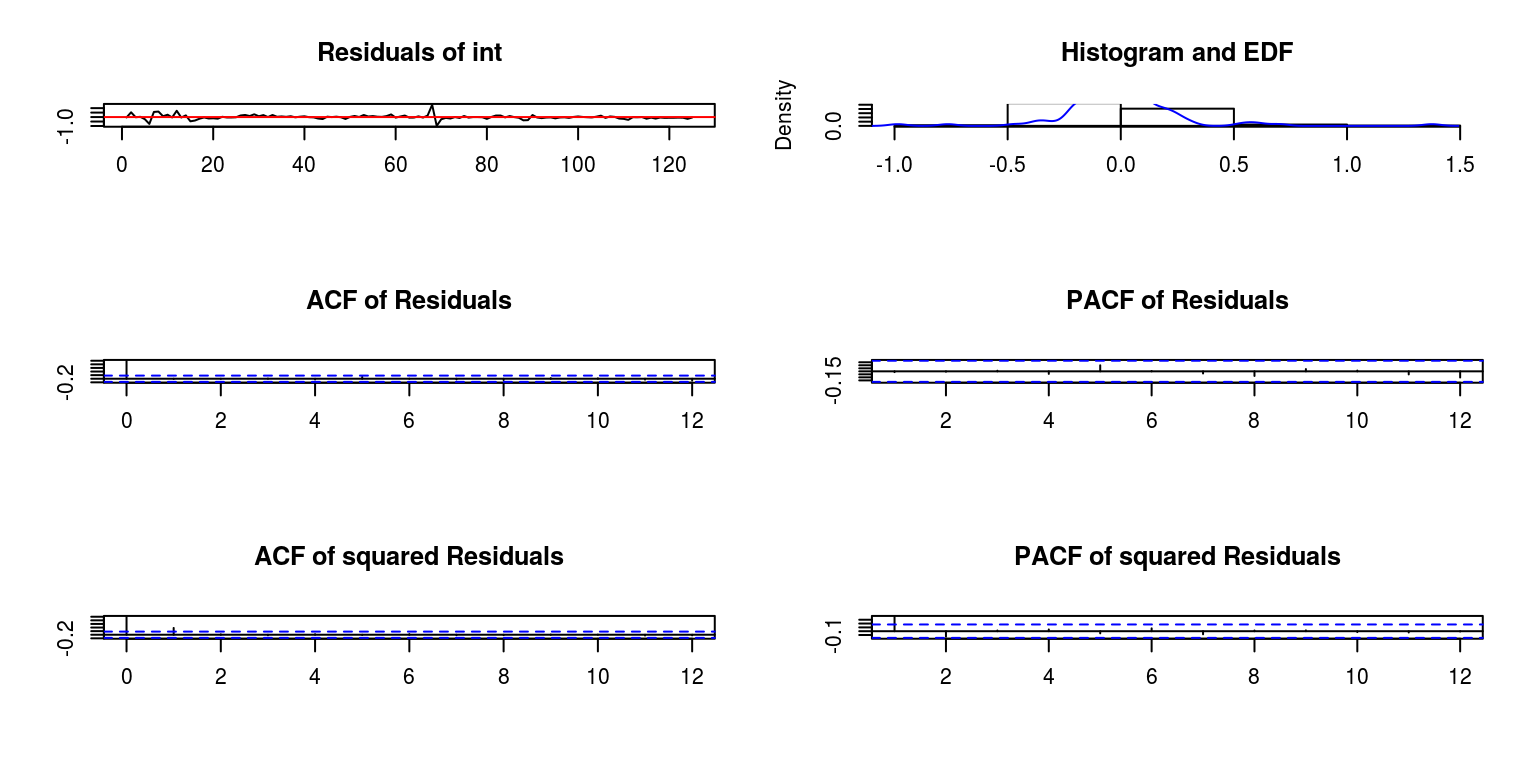``plot(bv.serial, names = "inf")``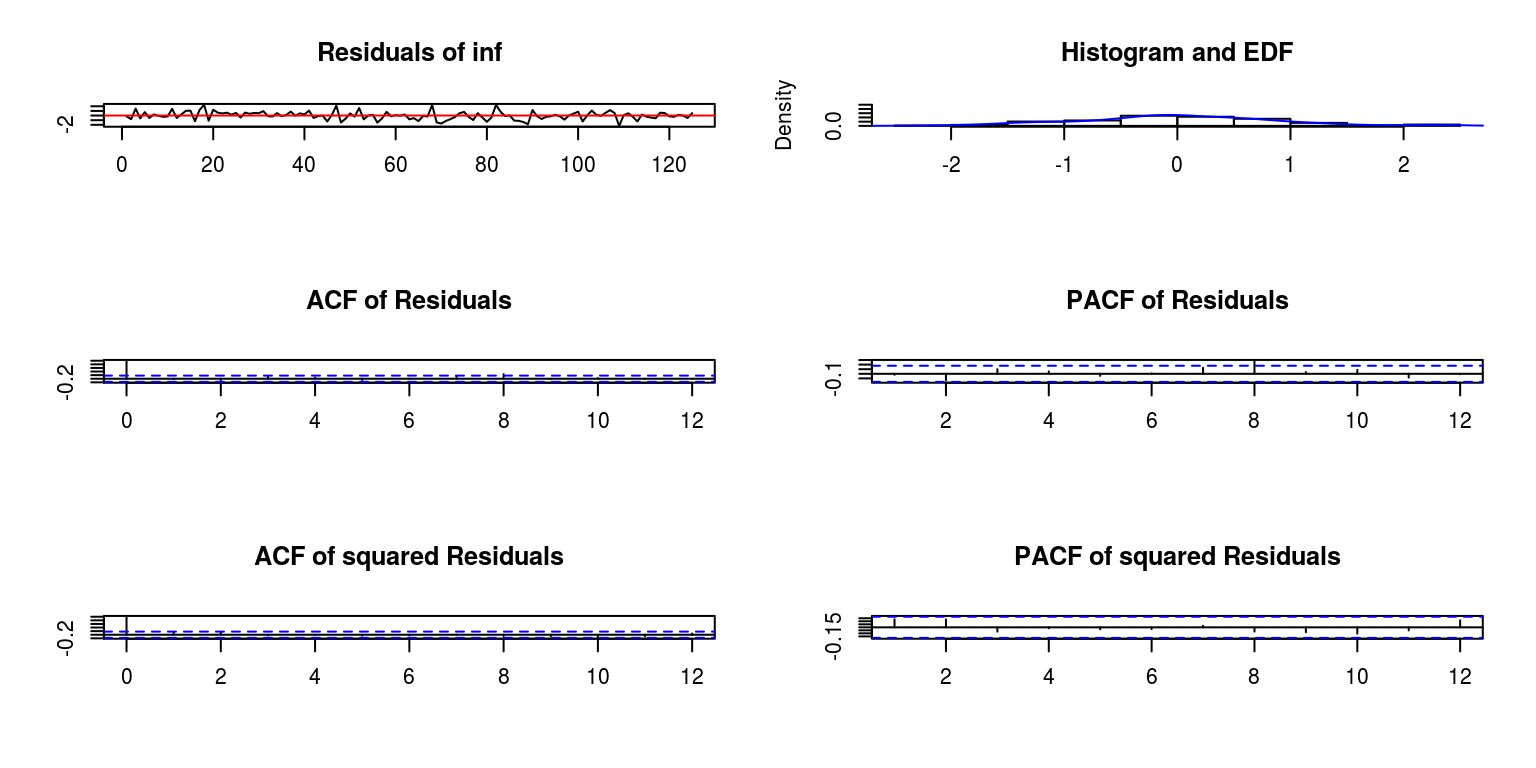To interpret these statistics note that a p-value that is greater than 5% would generally indicate that there is an absence of serial correlation. To test for heteroscedasticity in the residuals we can perform a multivariate ARCH Lagrange-Multiplier test.

``````bv.arch <- arch.test(bv.est, lags.multi = 12, multivariate.only = TRUE)
bv.arch``````
``````##
##  ARCH (multivariate)
##
## data:  Residuals of VAR object bv.est
## Chi-squared = 133.27, df = 108, p-value =
## 0.04992``````

Once again the p-value that is greater than 5% would indicate the absence of heteroscedasticity. To consider the distribution of the residuals, we could apply a normality test.

``````bv.norm <- normality.test(bv.est, multivariate.only = TRUE)
bv.norm``````
``````## \$JB
##
##  JB-Test (multivariate)
##
## data:  Residuals of VAR object bv.est
## Chi-squared = 574.06, df = 4, p-value < 2.2e-16
##
##
## \$Skewness
##
##  Skewness only (multivariate)
##
## data:  Residuals of VAR object bv.est
## Chi-squared = 25.135, df = 2, p-value =
## 3.483e-06
##
##
## \$Kurtosis
##
##  Kurtosis only (multivariate)
##
## data:  Residuals of VAR object bv.est
## Chi-squared = 548.93, df = 2, p-value < 2.2e-16``````

where the resulting p-value that there could be a problem with skewness and kurtosis, which is mainly relate to interest rates (big error around observation 70). We could then use dummies for these outliers, which would be included as exogenous variable

``````resid <- residuals(bv.est)
par(mfrow = c(1, 1))
plot.ts(resid[, 1])``````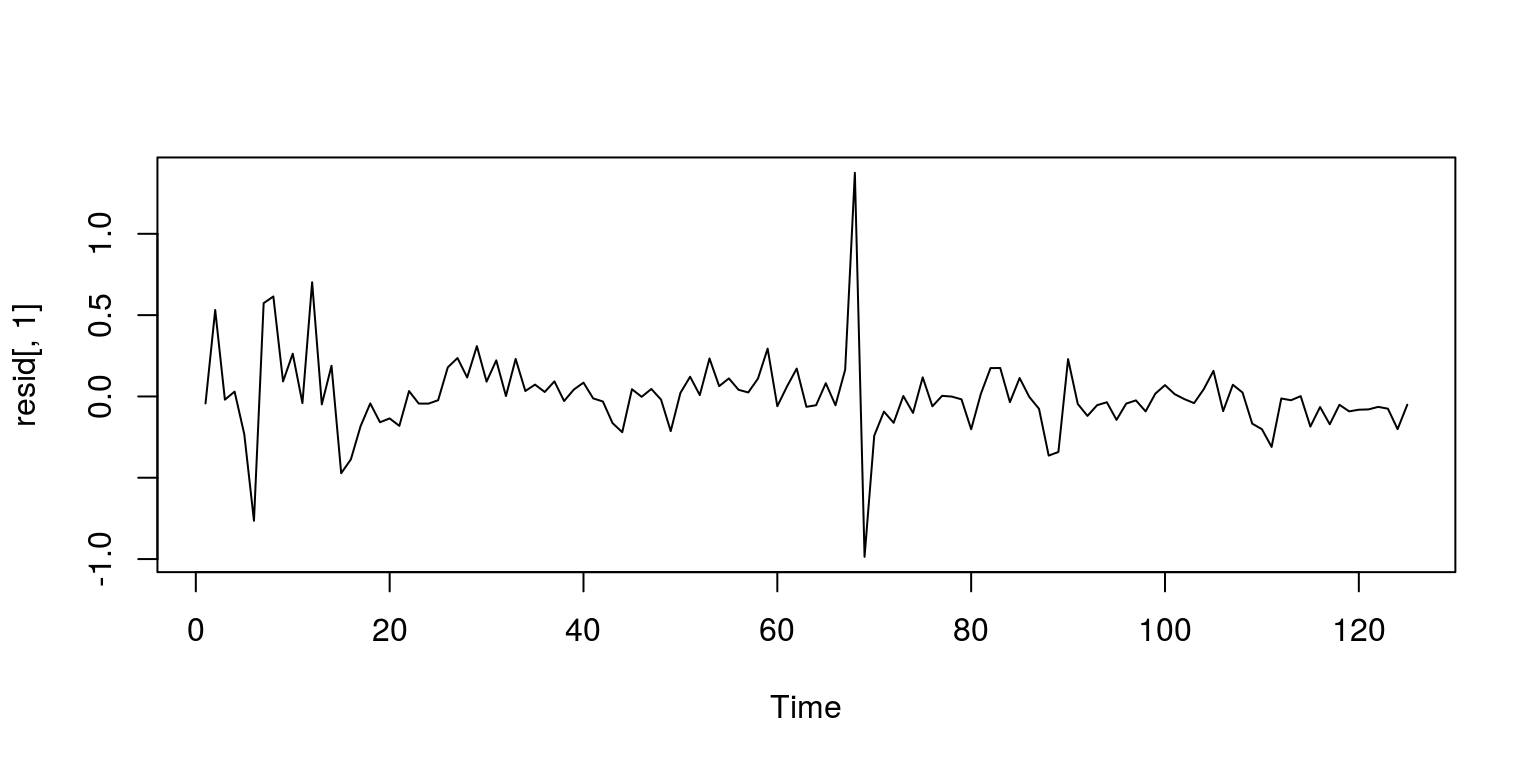``plot.ts(resid[60:80, 1])``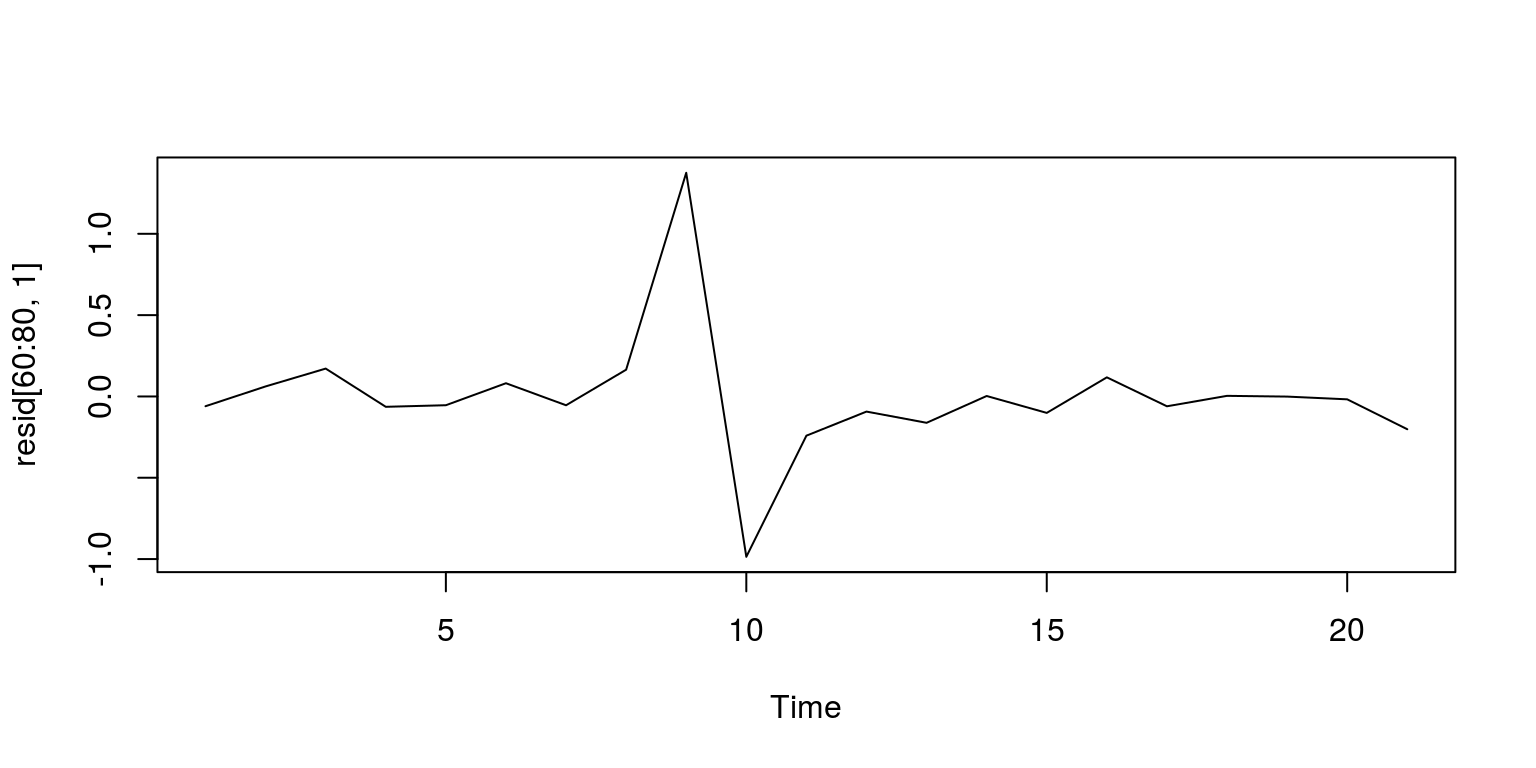``resid[60:80, 1]``
``````##            60            61            62
## -0.0601291310  0.0615441868  0.1712033745
##            63            64            65
## -0.0637818003 -0.0540271760  0.0812039374
##            66            67            68
## -0.0542655393  0.1643875680  1.3748496180
##            69            70            71
## -0.9858961840 -0.2412059245 -0.0931490801
##            72            73            74
## -0.1621170496  0.0027209500 -0.1011986223
##            75            76            77
##  0.1173297464 -0.0605540326  0.0036229021
##            78            79            80
## -0.0009667933 -0.0178211827 -0.2017696513``````
``````dum <- rep(0, dim(dat.bv))
dum <- 1
dum <- 1
plot(dum)``````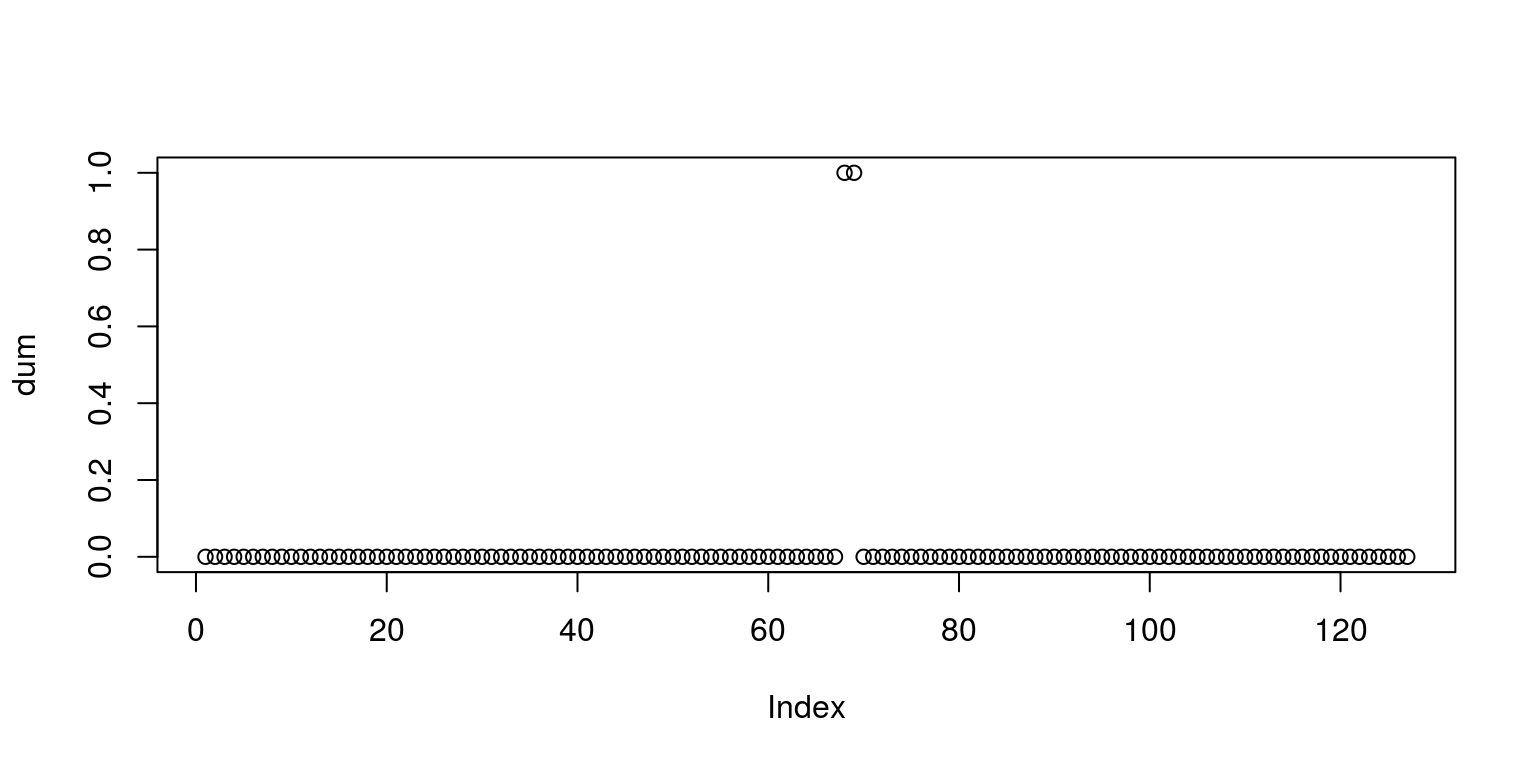``````bv.estdum <- VAR(dat.bv, p = 2, type = "const", season = NULL,
exog = dum)
bv.serialdum <- serial.test(bv.estdum, lags.pt = 12, type = "PT.asymptotic")
plot(bv.serialdum)``````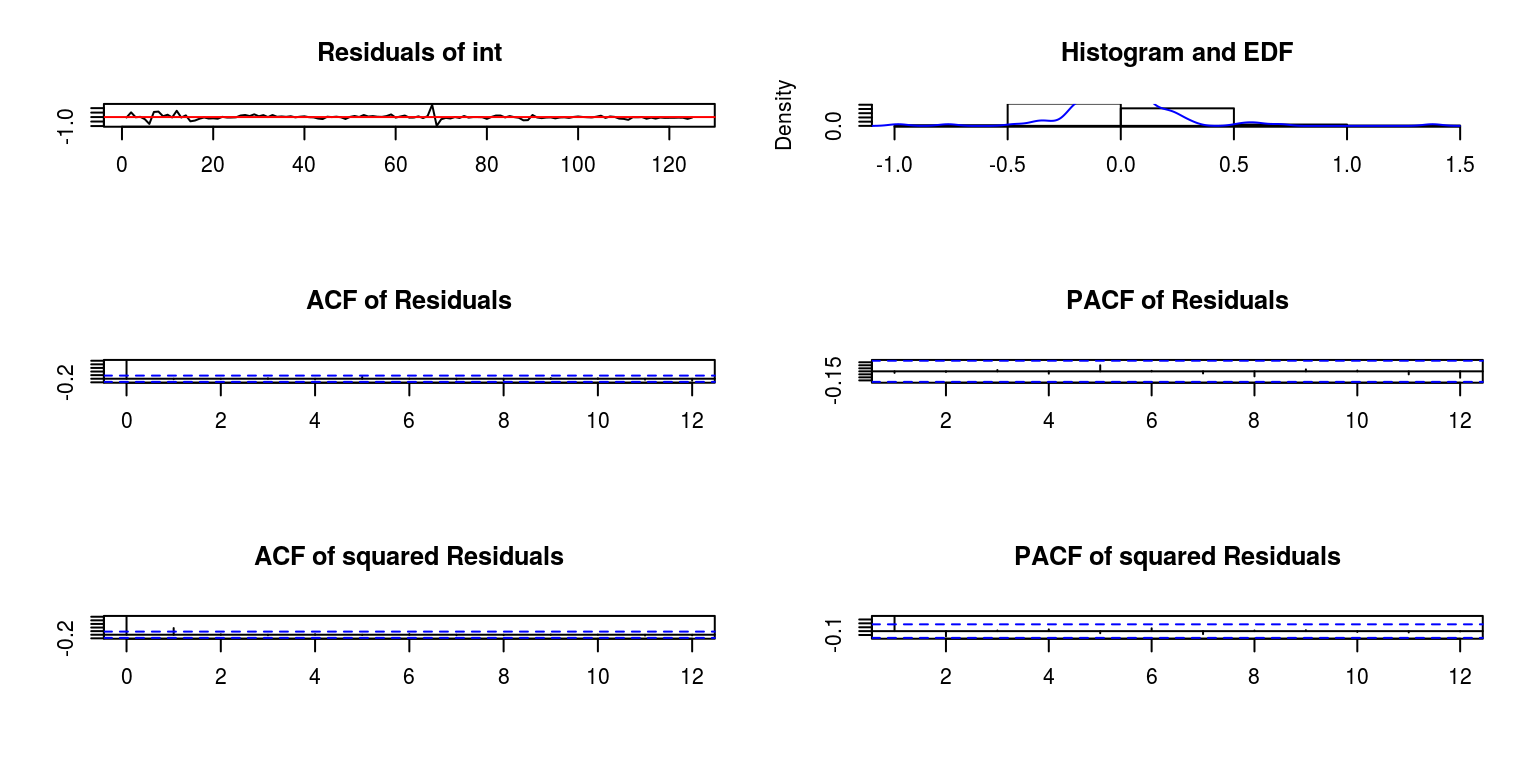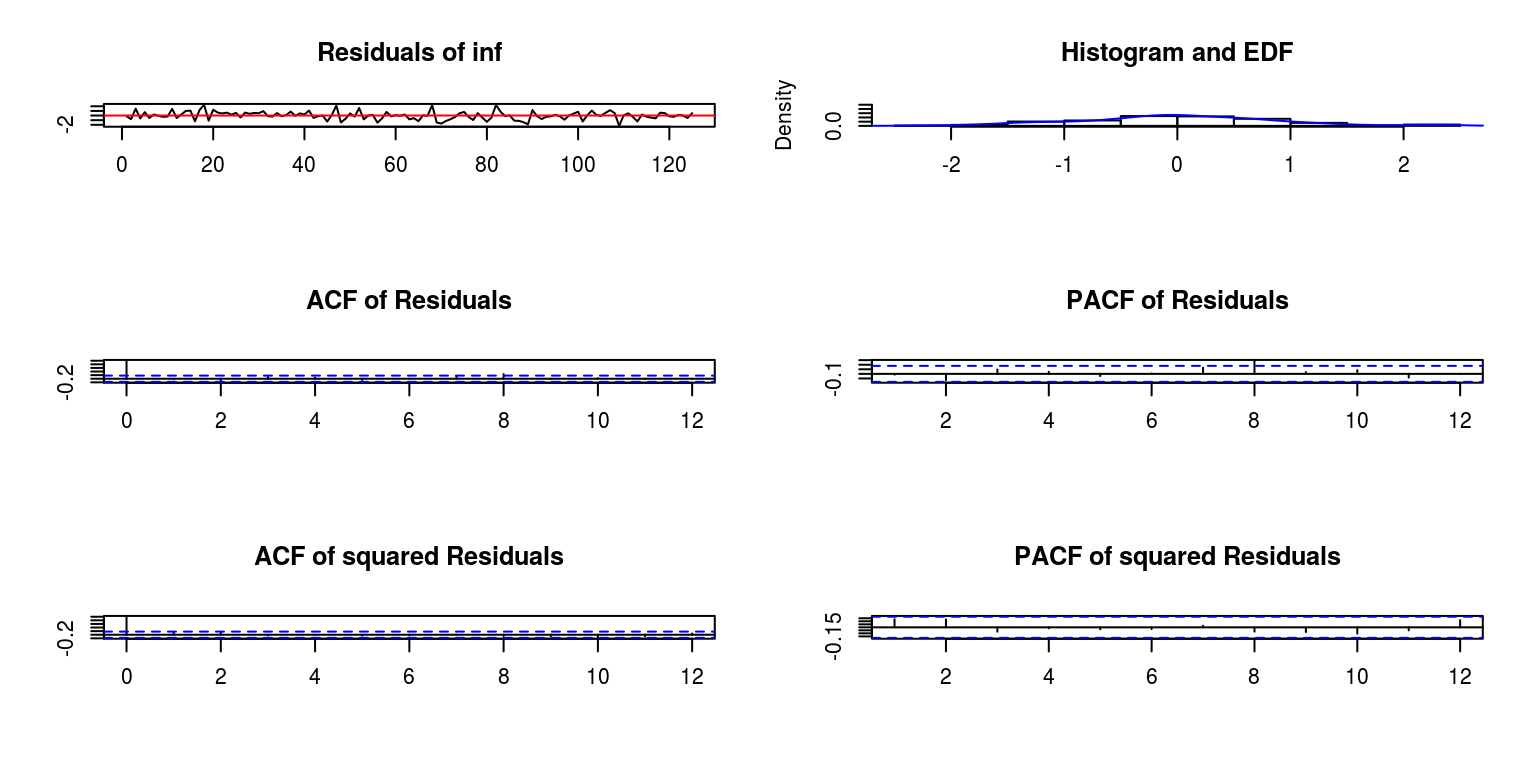``````bv.normdum <- normality.test(bv.estdum, multivariate.only = TRUE)
bv.normdum``````
``````## \$JB
##
##  JB-Test (multivariate)
##
## data:  Residuals of VAR object bv.estdum
## Chi-squared = 583.3, df = 4, p-value < 2.2e-16
##
##
## \$Skewness
##
##  Skewness only (multivariate)
##
## data:  Residuals of VAR object bv.estdum
## Chi-squared = 25.912, df = 2, p-value =
## 2.362e-06
##
##
## \$Kurtosis
##
##  Kurtosis only (multivariate)
##
## data:  Residuals of VAR object bv.estdum
## Chi-squared = 557.39, df = 2, p-value < 2.2e-16``````

Unfortunately, this makes very little difference. To test for structural stability, we apply the CUSUM test as follows:

``````bv.cusum <- stability(bv.est, type = "OLS-CUSUM")
plot(bv.cusum)``````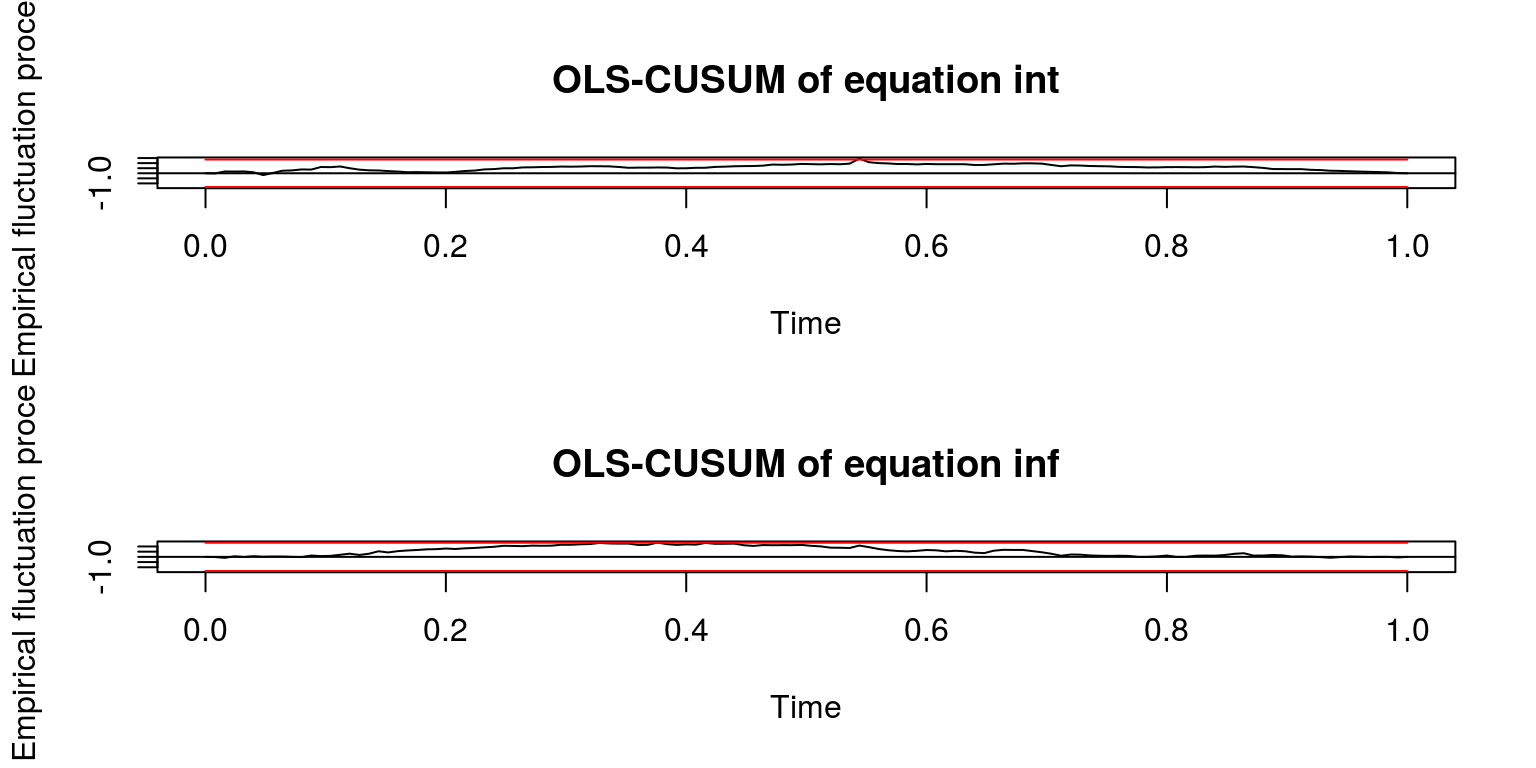which seems to be more or less ok?

## 2.2 Granger causality, IRFs and variance decompositions

We are then able to test for Granger causality, where we note that the null hypothesis of no Granger causality is dismissed in both directions.

``````bv.cause.int <- causality(bv.est, cause = "int")
bv.cause.int``````
``````## \$Granger
##
##  Granger causality H0: int do not Granger-cause
##  inf
##
## data:  VAR object bv.est
## F-Test = 1.296, df1 = 2, df2 = 240, p-value =
## 0.2755
##
##
## \$Instant
##
##  H0: No instantaneous causality between: int and
##  inf
##
## data:  VAR object bv.est
## Chi-squared = 10.72, df = 1, p-value = 0.00106``````
``````bv.cause.inf <- causality(bv.est, cause = "inf")
bv.cause.inf``````
``````## \$Granger
##
##  Granger causality H0: inf do not Granger-cause
##  int
##
## data:  VAR object bv.est
## F-Test = 0.77066, df1 = 2, df2 = 240, p-value =
## 0.4639
##
##
## \$Instant
##
##  H0: No instantaneous causality between: inf and
##  int
##
## data:  VAR object bv.est
## Chi-squared = 10.72, df = 1, p-value = 0.00106``````

To generate impulse response functions to describe the reponse of interest rate to other shocks, we proceed as follows:

``````irf.int <- irf(bv.est, response = "int", n.ahead = 40, boot = TRUE)
plot(irf.int)``````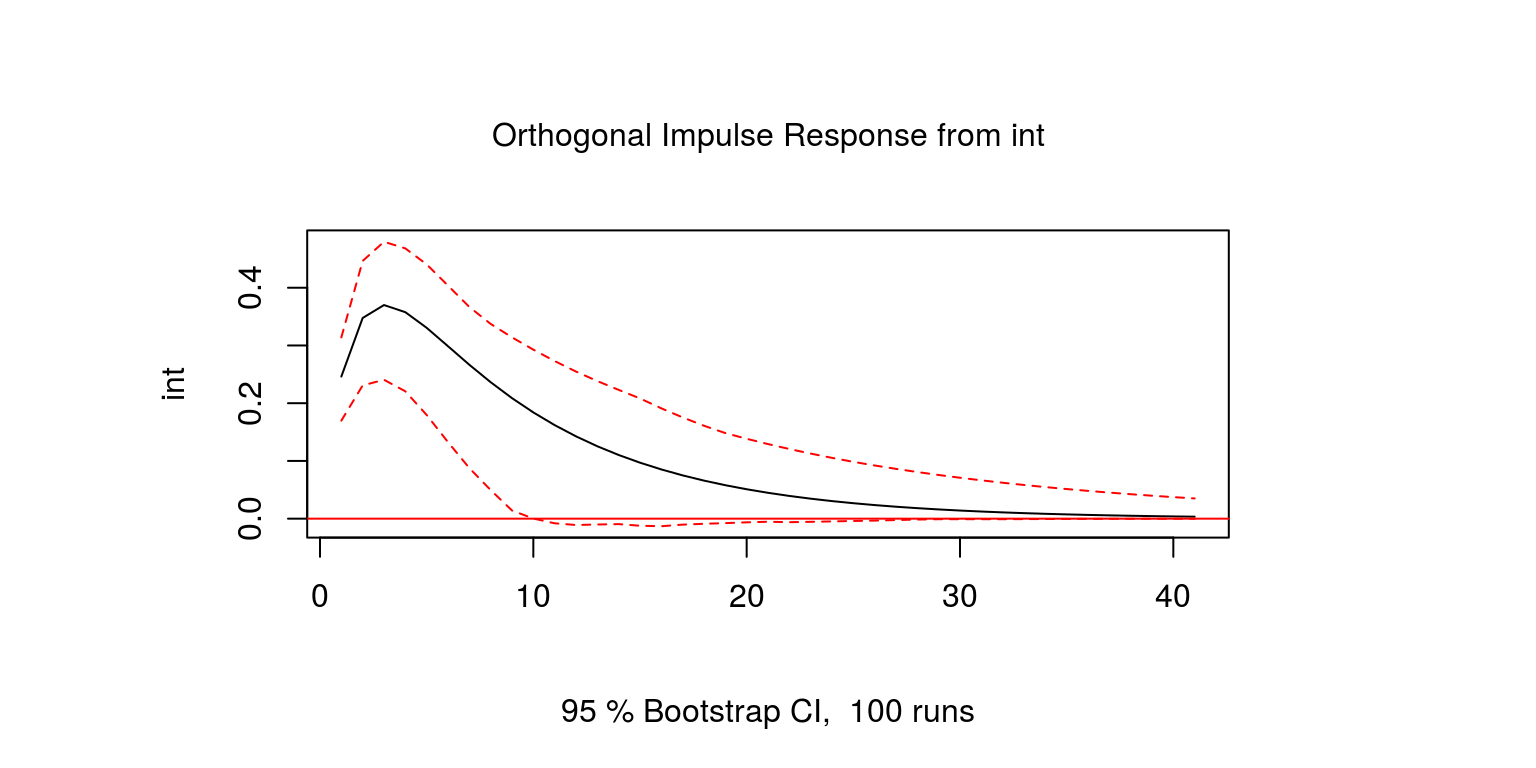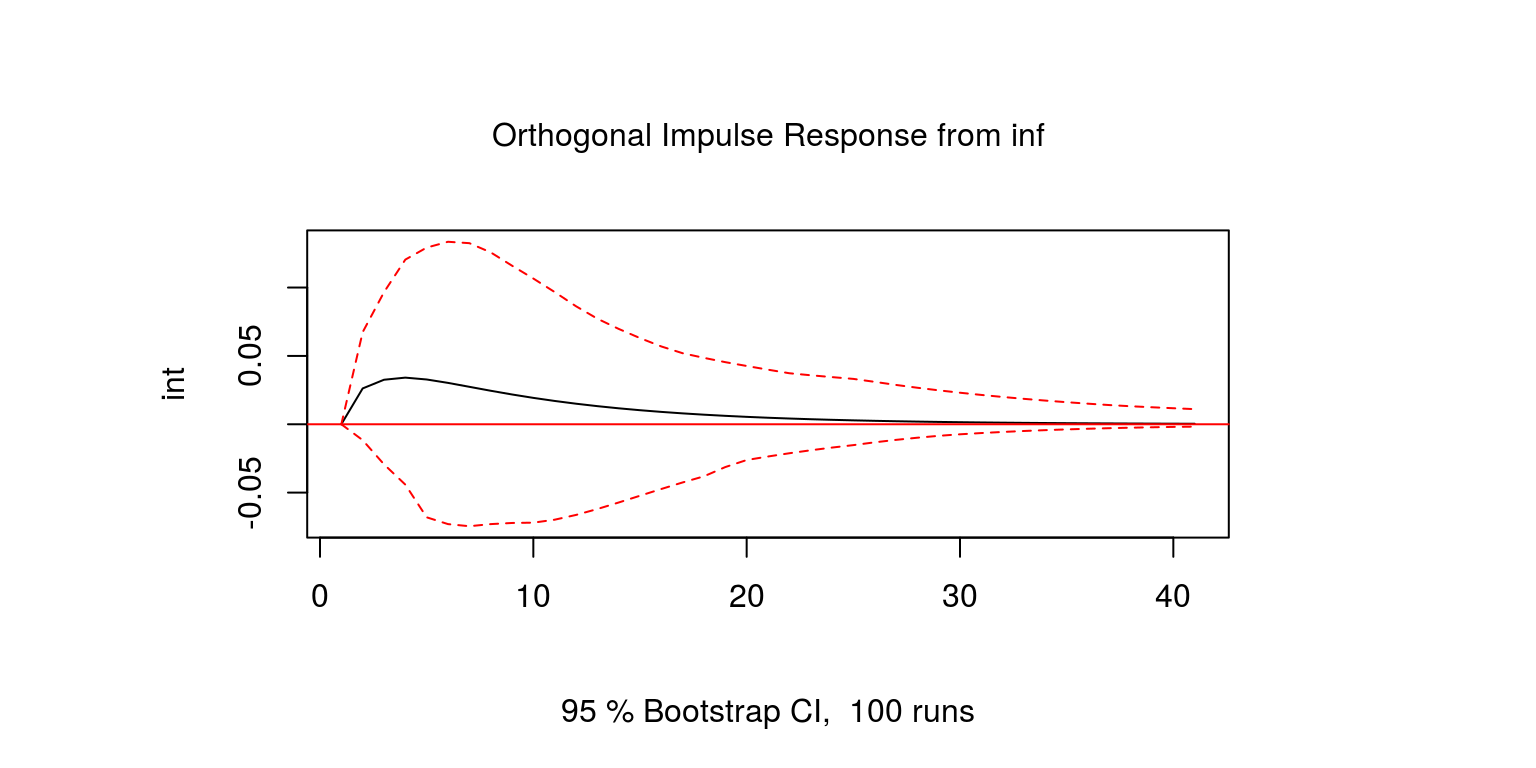Similarly, to consider the reponse of reponse of inflation to other shocks:

``````irf.inf <- irf(bv.est, response = "inf", n.ahead = 40, boot = TRUE)
plot(irf.inf)``````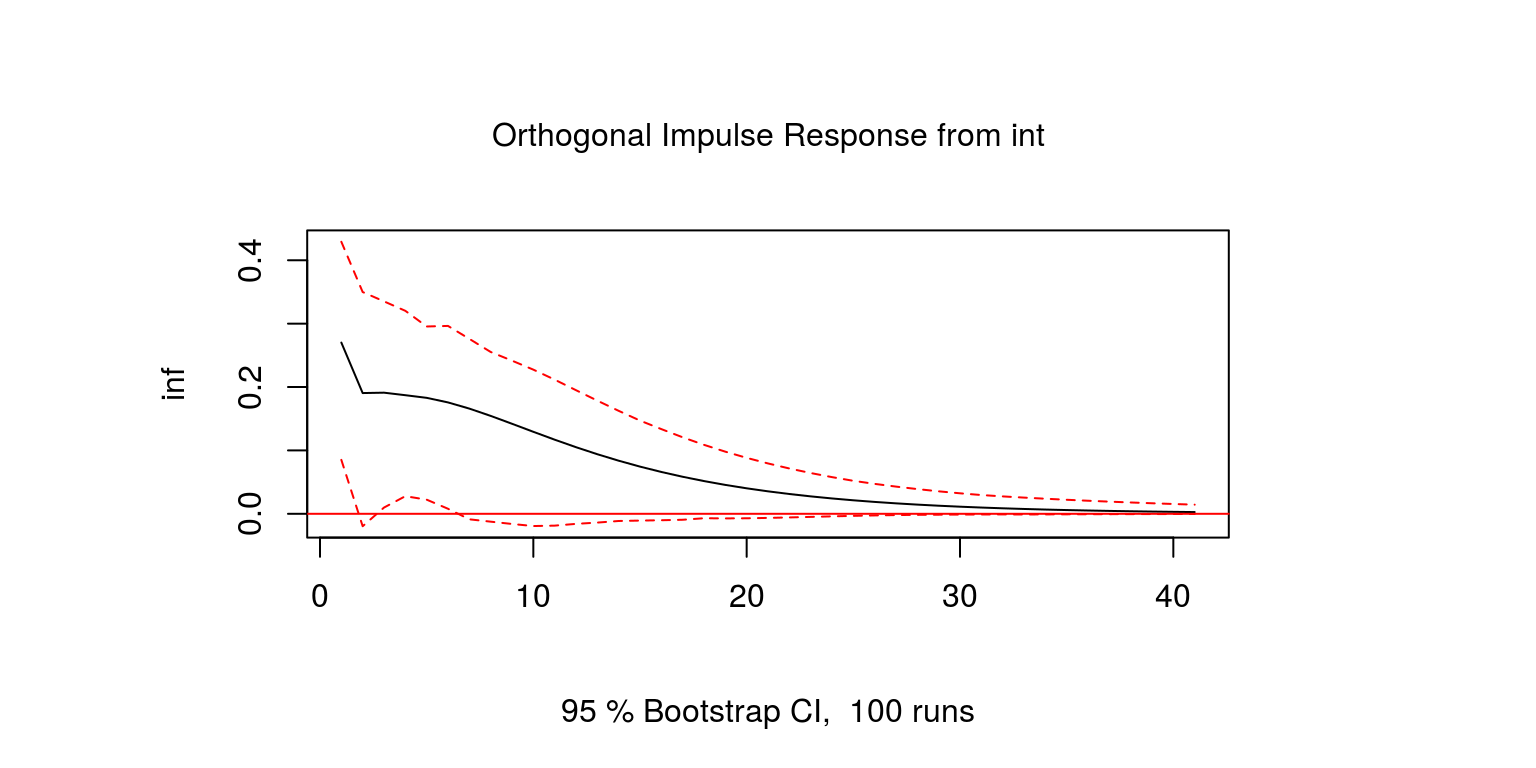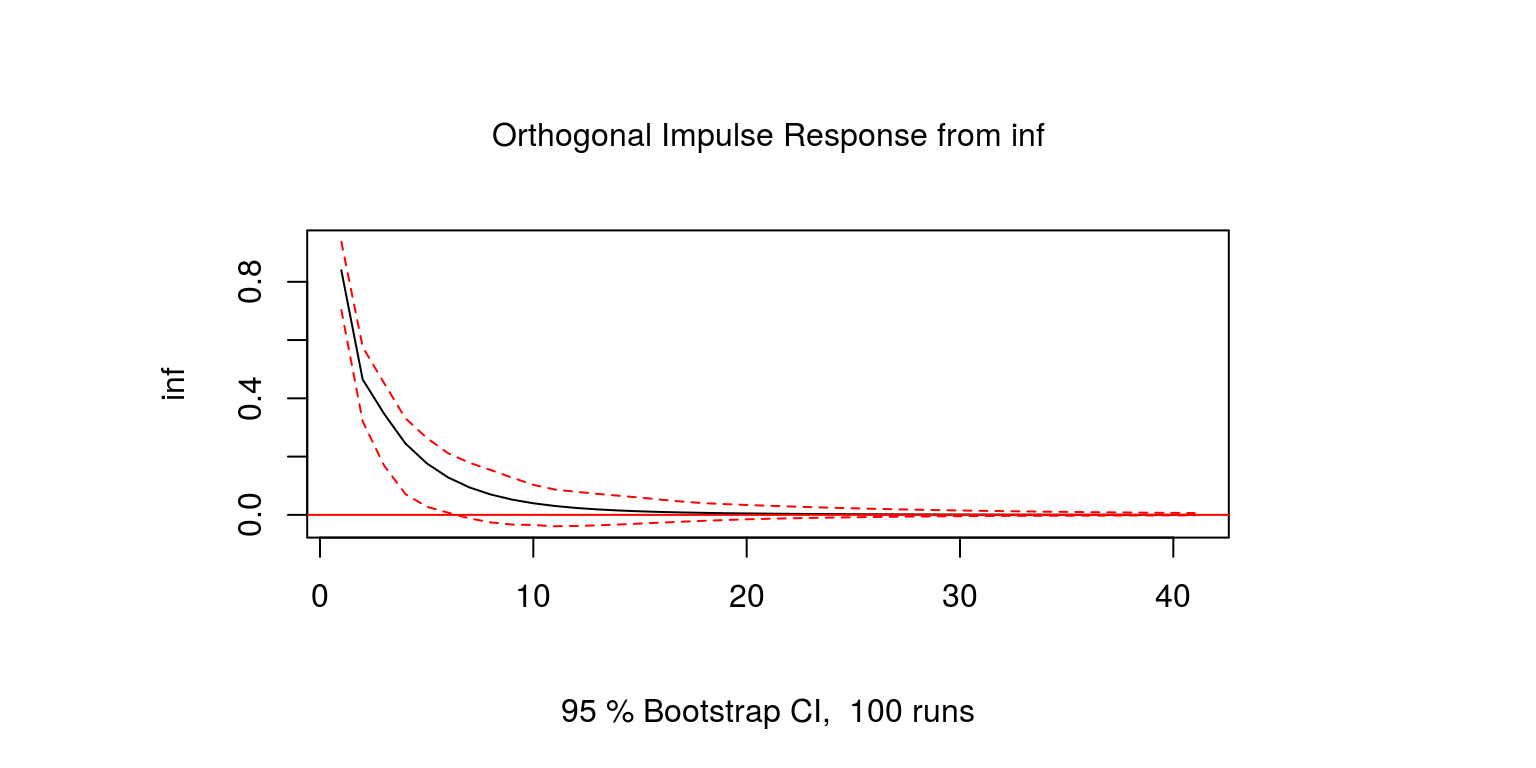This is not what we wanted to see and would suggest that there is some evidence of the Price puzzle. It may be that the dynamics may be more involved than a standard VAR allows, or we may need more than two variables.

To generate the forecast error variance decompositions.

``````bv.vardec <- fevd(bv.est, n.ahead = 10)
plot(bv.vardec)``````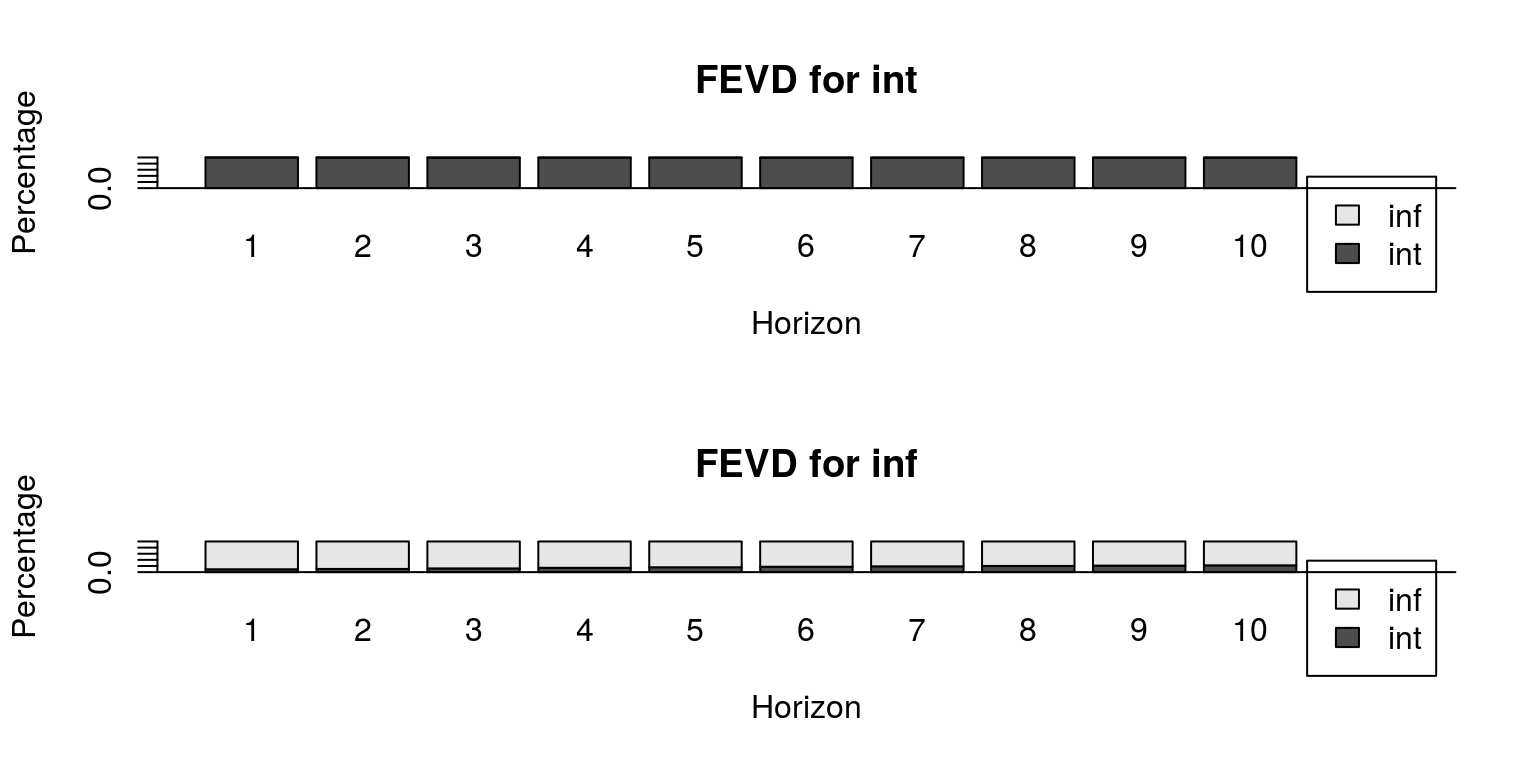where we note that interest rates are really only determined by interest rate shocks, while inflation is influenced by interest rate shocks to a certain degree.